# US10225123B2 - Method of signal generation and signal generating device - Google Patents

## Info

Publication number
US10225123B2
US10225123B2 US15/987,016 US201815987016A US10225123B2 US 10225123 B2 US10225123 B2 US 10225123B2 US 201815987016 A US201815987016 A US 201815987016A US 10225123 B2 US10225123 B2 US 10225123B2
Authority
US
United States
Prior art keywords
phase
signal
modulation scheme
change
scheme
Prior art date
Legal status (The legal status is an assumption and is not a legal conclusion. Google has not performed a legal analysis and makes no representation as to the accuracy of the status listed.)
Active
Application number
US15/987,016
Other versions
US20180270096A1 (en
Inventor
Yutaka Murakami
Tomohiro Kimura
Mikihiro Ouchi
Current Assignee (The listed assignees may be inaccurate. Google has not performed a legal analysis and makes no representation or warranty as to the accuracy of the list.)
Sun Patent Trust
Original Assignee
Sun Patent Trust
Priority date (The priority date is an assumption and is not a legal conclusion. Google has not performed a legal analysis and makes no representation as to the accuracy of the date listed.)
Filing date
Publication date
Priority to JP2011-033771 priority Critical
Priority to JP2011033771 priority
Priority to JP2011-051842 priority
Priority to JP2011051842 priority
Priority to JP2011-093544 priority
Priority to JP2011093544 priority
Priority to JP2011102101 priority
Priority to JP2011-102101 priority
Priority to PCT/JP2012/000352 priority patent/WO2012111256A1/en
Priority to US201313811064A priority
Priority to US14/501,780 priority patent/US9667333B2/en
Priority to US15/496,406 priority patent/US10009207B2/en
Priority to US15/987,016 priority patent/US10225123B2/en
Application filed by Sun Patent Trust filed Critical Sun Patent Trust
Publication of US20180270096A1 publication Critical patent/US20180270096A1/en
Application granted granted Critical
Publication of US10225123B2 publication Critical patent/US10225123B2/en
Application status is Active legal-status Critical
Anticipated expiration legal-status Critical

• 230000007274 generation of a signal involved in cell-cell signaling Effects 0 claims description title 7
• 230000000051 modifying Effects 0 abstract description 1354
• 239000011159 matrix materials Substances 0 abstract description 106
• 230000001603 reducing Effects 0 claims description 19
• 238000006722 reduction reaction Methods 0 claims description 16
• 239000000969 carrier Substances 0 description 288
• 230000000875 corresponding Effects 0 description 98
• 238000004891 communication Methods 0 description 67
• 239000011589 pyridoxal 5'-phosphate Substances 0 description 59
• 230000000694 effects Effects 0 description 47
• 230000001976 improved Effects 0 description 32
• 238000006243 chemical reaction Methods 0 description 27
• 230000003321 amplification Effects 0 description 22
• 238000003199 nucleic acid amplification method Methods 0 description 22
• 238000003780 insertion Methods 0 description 21
• 239000002609 media Substances 0 description 19
• 102100015331 MTA2 Human genes 0 description 16
• 101700084861 MTA2 family Proteins 0 description 16
• 101700073430 PID family Proteins 0 description 16
• 239000000284 extracts Substances 0 description 16
• 230000001629 suppression Effects 0 description 16
• 230000015556 catabolic process Effects 0 description 15
• 238000006731 degradation Methods 0 description 15
• 230000004059 degradation Effects 0 description 15
• 238000004364 calculation methods Methods 0 description 14
• 238000006011 modification Methods 0 description 13
• 230000004048 modification Effects 0 description 13
• 238000005516 engineering processes Methods 0 description 11
• 210000002683 Foot Anatomy 0 description 10
• 239000004695 Polyether sulfone Substances 0 description 10
• 229920002496 poly(ether sulfone) Polymers 0 description 10
• 229920003208 poly(ethylene sulfide) Polymers 0 description 10
• 239000000047 products Substances 0 description 10
• 230000002829 reduced Effects 0 description 10
• 238000001228 spectrum Methods 0 description 10
• 230000001419 dependent Effects 0 description 8
• 238000009826 distribution Methods 0 description 8
• 230000015654 memory Effects 0 description 8
• 238000003786 synthesis Methods 0 description 8
• 230000001702 transmitter Effects 0 description 8
• 230000002452 interceptive Effects 0 description 7
• 238000000034 methods Methods 0 description 7
• 230000000737 periodic Effects 0 description 7
• 241000193803 Therea Species 0 description 6
• 238000004422 calculation algorithm Methods 0 description 6
• 230000011664 signaling Effects 0 description 6
• 238000007906 compression Methods 0 description 5
• 238000005562 fading Methods 0 description 5
• 239000011133 lead Substances 0 description 5
• 239000004065 semiconductor Substances 0 description 5
• 239000000203 mixtures Substances 0 description 4
• 230000001965 increased Effects 0 description 3
• 230000003287 optical Effects 0 description 3
• 238000000926 separation method Methods 0 description 3
• 238000003860 storage Methods 0 description 3
• 238000007792 addition Methods 0 description 2
• 238000000354 decomposition Methods 0 description 2
• 230000001934 delay Effects 0 description 2
• 238000009432 framing Methods 0 description 2
• 239000010410 layers Substances 0 description 2
• 239000003607 modifier Substances 0 description 2
• 239000003638 reducing agent Substances 0 description 2
• 102100012018 HSPG2 Human genes 0 description 1
• 101700050209 PELC family Proteins 0 description 1
• 101700018992 PGBM family Proteins 0 description 1
• 101700037860 PHLC family Proteins 0 description 1
• 101700082424 PHLC1 family Proteins 0 description 1
• 101700056117 PHLD family Proteins 0 description 1
• 101700005116 PLC family Proteins 0 description 1
• 101700019706 PLY3 family Proteins 0 description 1
• 230000002730 additional Effects 0 description 1
• 238000004458 analytical methods Methods 0 description 1
• 238000005047 biotechnology Methods 0 description 1
• 230000001721 combination Effects 0 description 1
• 230000001276 controlling effects Effects 0 description 1
• 238000005265 energy consumption Methods 0 description 1
• 239000002529 flux Substances 0 description 1
• 230000014509 gene expression Effects 0 description 1
• 230000001788 irregular Effects 0 description 1
• 230000000670 limiting Effects 0 description 1
• 238000005457 optimization Methods 0 description 1
• 230000036961 partial Effects 0 description 1
• 230000003405 preventing Effects 0 description 1
• 238000003672 processing method Methods 0 description 1
• 230000004044 response Effects 0 description 1
• 238000005070 sampling Methods 0 description 1
• 238000004088 simulation Methods 0 description 1
• 239000007787 solids Substances 0 description 1
• 230000001502 supplementation Effects 0 description 1
• 230000001360 synchronised Effects 0 description 1
• 238000000844 transformation Methods 0 description 1
• 230000001131 transforming Effects 0 description 1

## Images

•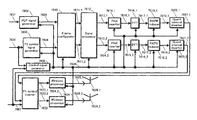•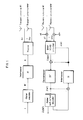•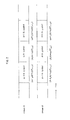•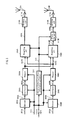•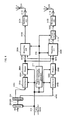•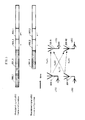•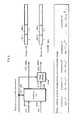•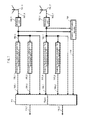•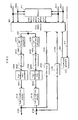•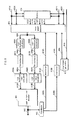•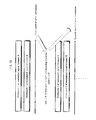•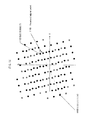•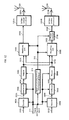•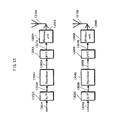•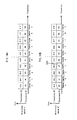•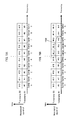•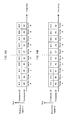•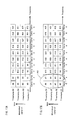••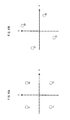••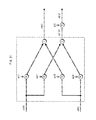•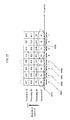•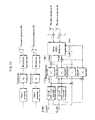••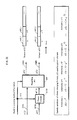•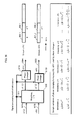•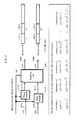•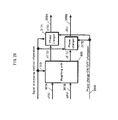•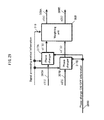•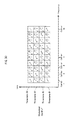••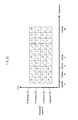•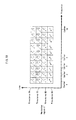•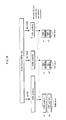•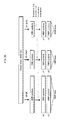•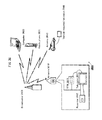•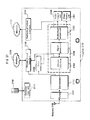•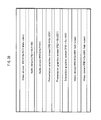•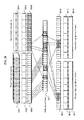•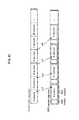•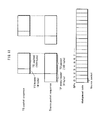•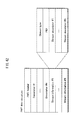•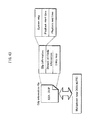•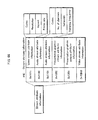•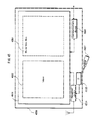•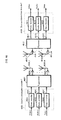•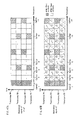•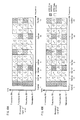•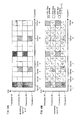•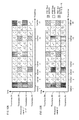•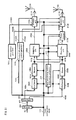•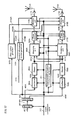•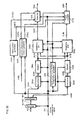•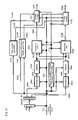•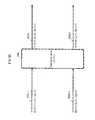•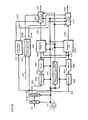•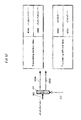•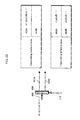•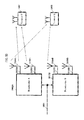•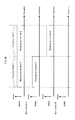•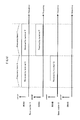•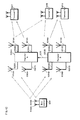•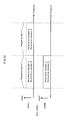•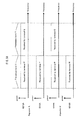•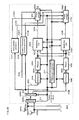•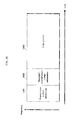•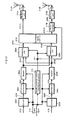•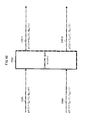•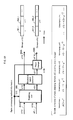•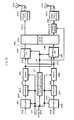•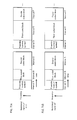•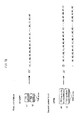••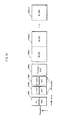•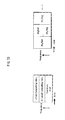•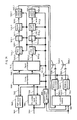•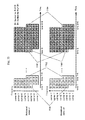•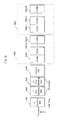•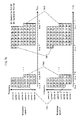•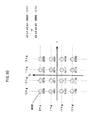•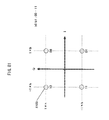•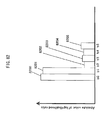•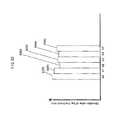•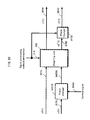•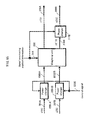•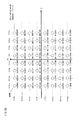•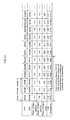••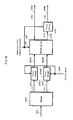•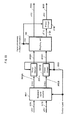•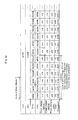•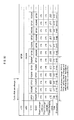•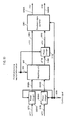•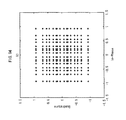•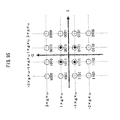## Classifications

• HELECTRICITY
• H04ELECTRIC COMMUNICATION TECHNIQUE
• H04LTRANSMISSION OF DIGITAL INFORMATION, e.g. TELEGRAPHIC COMMUNICATION
• H04L27/00Modulated-carrier systems
• H04L27/26Systems using multi-frequency codes
• H04L27/2601Multicarrier modulation systems
• H04L27/2626Arrangements specific to the transmitter
• H04L27/2627Modulators
• H04L27/2628Inverse Fourier transform modulators, e.g. IFFT/IDFT
• HELECTRICITY
• H04ELECTRIC COMMUNICATION TECHNIQUE
• H04BTRANSMISSION
• H04B7/02Diversity systems; Multi-antenna system, i.e. transmission or reception using multiple antennas
• H04B7/04Diversity systems; Multi-antenna system, i.e. transmission or reception using multiple antennas using two or more spaced independent antennas
• H04B7/06Diversity systems; Multi-antenna system, i.e. transmission or reception using multiple antennas using two or more spaced independent antennas at the transmitting station
• H04B7/0613Diversity systems; Multi-antenna system, i.e. transmission or reception using multiple antennas using two or more spaced independent antennas at the transmitting station using simultaneous transmission
• H04B7/0667Diversity systems; Multi-antenna system, i.e. transmission or reception using multiple antennas using two or more spaced independent antennas at the transmitting station using simultaneous transmission of delayed versions of same signal
• HELECTRICITY
• H04ELECTRIC COMMUNICATION TECHNIQUE
• H04BTRANSMISSION
• H04B7/02Diversity systems; Multi-antenna system, i.e. transmission or reception using multiple antennas
• H04B7/04Diversity systems; Multi-antenna system, i.e. transmission or reception using multiple antennas using two or more spaced independent antennas
• H04B7/0413MIMO systems
• HELECTRICITY
• H04ELECTRIC COMMUNICATION TECHNIQUE
• H04BTRANSMISSION
• H04B7/02Diversity systems; Multi-antenna system, i.e. transmission or reception using multiple antennas
• H04B7/04Diversity systems; Multi-antenna system, i.e. transmission or reception using multiple antennas using two or more spaced independent antennas
• H04B7/06Diversity systems; Multi-antenna system, i.e. transmission or reception using multiple antennas using two or more spaced independent antennas at the transmitting station
• H04B7/0613Diversity systems; Multi-antenna system, i.e. transmission or reception using multiple antennas using two or more spaced independent antennas at the transmitting station using simultaneous transmission
• H04B7/0682Diversity systems; Multi-antenna system, i.e. transmission or reception using multiple antennas using two or more spaced independent antennas at the transmitting station using simultaneous transmission using phase diversity (e.g. phase sweeping)
• HELECTRICITY
• H04ELECTRIC COMMUNICATION TECHNIQUE
• H04BTRANSMISSION
• H04B7/02Diversity systems; Multi-antenna system, i.e. transmission or reception using multiple antennas
• H04B7/04Diversity systems; Multi-antenna system, i.e. transmission or reception using multiple antennas using two or more spaced independent antennas
• H04B7/06Diversity systems; Multi-antenna system, i.e. transmission or reception using multiple antennas using two or more spaced independent antennas at the transmitting station
• H04B7/0697Diversity systems; Multi-antenna system, i.e. transmission or reception using multiple antennas using two or more spaced independent antennas at the transmitting station using spatial multiplexing
• HELECTRICITY
• H04ELECTRIC COMMUNICATION TECHNIQUE
• H04BTRANSMISSION
• H04B7/02Diversity systems; Multi-antenna system, i.e. transmission or reception using multiple antennas
• H04B7/04Diversity systems; Multi-antenna system, i.e. transmission or reception using multiple antennas using two or more spaced independent antennas
• H04B7/08Diversity systems; Multi-antenna system, i.e. transmission or reception using multiple antennas using two or more spaced independent antennas at the receiving station
• H04B7/0837Diversity systems; Multi-antenna system, i.e. transmission or reception using multiple antennas using two or more spaced independent antennas at the receiving station using pre-detection combining
• H04B7/0842Weighted combining
• HELECTRICITY
• H04ELECTRIC COMMUNICATION TECHNIQUE
• H04LTRANSMISSION OF DIGITAL INFORMATION, e.g. TELEGRAPHIC COMMUNICATION
• H04L1/00Arrangements for detecting or preventing errors in the information received
• H04L1/004Arrangements for detecting or preventing errors in the information received by using forward error control
• H04L1/0045Arrangements at the receiver end
• H04L1/0047Decoding adapted to other signal detection operation
• H04L1/0048Decoding adapted to other signal detection operation in conjunction with detection of multiuser or interfering signals, e.g. iteration between CDMA or MIMO detector and FEC decoder
• HELECTRICITY
• H04ELECTRIC COMMUNICATION TECHNIQUE
• H04LTRANSMISSION OF DIGITAL INFORMATION, e.g. TELEGRAPHIC COMMUNICATION
• H04L27/00Modulated-carrier systems
• H04L27/18Phase-modulated carrier systems, i.e. using phase-shift keying includes continuous phase systems
• H04L27/20Modulator circuits; Transmitter circuits
• HELECTRICITY
• H04ELECTRIC COMMUNICATION TECHNIQUE
• H04LTRANSMISSION OF DIGITAL INFORMATION, e.g. TELEGRAPHIC COMMUNICATION
• H04L27/00Modulated-carrier systems
• H04L27/26Systems using multi-frequency codes
• H04L27/2601Multicarrier modulation systems
• H04L27/2647Arrangements specific to the receiver
• H04L27/2649Demodulators
• H04L27/265Fourier transform demodulators
• HELECTRICITY
• H04ELECTRIC COMMUNICATION TECHNIQUE
• H04LTRANSMISSION OF DIGITAL INFORMATION, e.g. TELEGRAPHIC COMMUNICATION
• H04L27/00Modulated-carrier systems
• H04L27/32Carrier systems characterised by combinations of two or more of the types covered by groups H04L27/02, H04L27/10, H04L27/18 or H04L27/26
• H04L27/34Amplitude- and phase-modulated carrier systems, e.g. quadrature-amplitude modulated carrier systems
• H04L27/36Modulator circuits; Transmitter circuits
• H04L27/366Arrangements for compensating undesirable properties of the transmission path between the modulator and the demodulator
• H04L27/367Arrangements for compensating undesirable properties of the transmission path between the modulator and the demodulator using predistortion
• H04L27/368Arrangements for compensating undesirable properties of the transmission path between the modulator and the demodulator using predistortion adaptive predistortion
• HELECTRICITY
• H04ELECTRIC COMMUNICATION TECHNIQUE
• H04LTRANSMISSION OF DIGITAL INFORMATION, e.g. TELEGRAPHIC COMMUNICATION
• H04L5/00Arrangements affording multiple use of the transmission path
• H04L5/0001Arrangements for dividing the transmission path
• H04L5/0014Three-dimensional division
• H04L5/0023Time-frequency-space
• HELECTRICITY
• H04ELECTRIC COMMUNICATION TECHNIQUE
• H04LTRANSMISSION OF DIGITAL INFORMATION, e.g. TELEGRAPHIC COMMUNICATION
• H04L5/00Arrangements affording multiple use of the transmission path
• H04L5/003Arrangements for allocating sub-channels of the transmission path
• H04L5/0078Timing of allocation
• H04L5/0082Timing of allocation at predetermined intervals
• HELECTRICITY
• H04ELECTRIC COMMUNICATION TECHNIQUE
• H04LTRANSMISSION OF DIGITAL INFORMATION, e.g. TELEGRAPHIC COMMUNICATION
• H04L5/00Arrangements affording multiple use of the transmission path
• H04L5/02Channels characterised by the type of signal
• H04L5/12Channels characterised by the type of signal the signals being represented by different phase modulations of a single carrier
• HELECTRICITY
• H04ELECTRIC COMMUNICATION TECHNIQUE
• H04LTRANSMISSION OF DIGITAL INFORMATION, e.g. TELEGRAPHIC COMMUNICATION
• H04L1/00Arrangements for detecting or preventing errors in the information received
• H04L1/004Arrangements for detecting or preventing errors in the information received by using forward error control
• H04L1/0056Systems characterized by the type of code used
• H04L1/0071Use of interleaving

## Abstract

A transmission method simultaneously transmitting a first modulated signal and a second modulated signal at a common frequency performs precoding on both signals using a fixed precoding matrix and regularly changes the phase of at least one of the signals, thereby improving received data signal quality for a reception device.

## Description

CROSS-REFERENCE TO RELATED APPLICATIONS

This application is a continuation of application Ser. No. 15/496,406, filed Apr. 25, 2017, now U.S. Pat. No. 10,009,207, which is a continuation of application Ser. No. 14/501,780, filed Sep. 30, 2014, now U.S. Pat. No. 9,667,333, which is a continuation of application Ser. No. 13/811,064, now U.S. Pat. No. 8,885,596, which is the National Stage of International Application No. PCT/JP2012/000352, filed Jan. 20, 2012, which is based on applications No. 2011-033771 filed Feb. 18, 2011, 2011-051842 filed Mar. 9, 2011, 2011-093544 filed Apr. 19, 2011, and 2011-102101 filed Apr. 28, 2011 in Japan. The entire disclosures of the above-identified applications, including the specification, drawings and claims are incorporated herein by reference in their entirety.

TECHNICAL FIELD

The present invention relates to a transmission device and a reception device for communication using multiple antennas.

BACKGROUND ART

A MIMO (Multiple-Input, Multiple-Output) system is an example of a conventional communication system using multiple antennas. In multi-antenna communication, of which the MIMO system is typical, multiple transmission signals are each modulated, and each modulated signal is simultaneously transmitted from a different antenna in order to increase the transmission speed of the data.

FIG. 23 illustrates a sample configuration of a transmission and reception device having two transmit antennas and two receive antennas, and using two transmit modulated signals (transmit streams). In the transmission device, encoded data are interleaved, the interleaved data are modulated, and frequency conversion and the like are performed to generate transmission signals, which are then transmitted from antennas. In this case, the scheme for simultaneously transmitting different modulated signals from different transmit antennas at the same time and on a common frequency is a spatial multiplexing MIMO system.

In this context, Patent Literature 1 suggests using a transmission device provided with a different interleaving pattern for each transmit antenna. That is, the transmission device from FIG. 23 should use two distinct interleaving patterns performed by two interleavers (πa and πb). As for the reception device, Non-Patent Literature 1 and Non-Patent Literature 2 describe improving reception quality by iteratively using soft values for the detection scheme (by the MIMO detector of FIG. 23).

As it happens, models of actual propagation environments in wireless communications include NLOS (Non Line-Of-Sight), typified by a Rayleigh fading environment is representative, and LOS (Line-Of-Sight), typified by a Rician fading environment. When the transmission device transmits a single modulated signal, and the reception device performs maximal ratio combination on the signals received by a plurality of antennas and then demodulates and decodes the resulting signals, excellent reception quality can be achieved in a LOS environment, in particular in an environment where the Rician factor is large. The Rician factor represents the received power of direct waves relative to the received power of scattered waves. However, depending on the transmission system (e.g., a spatial multiplexing MIMO system), a problem occurs in that the reception quality deteriorates as the Rician factor increases (see Non-Patent Literature 3).

FIGS. 24A and 24B illustrate an example of simulation results of the BER (Bit Error Rate) characteristics (vertical axis: BER, horizontal axis: SNR (signal-to-noise ratio) for data encoded with LDPC (low-density parity-check) codes and transmitted over a 2×2 (two transmit antennas, two receive antennas) spatial multiplexing MIMO system in a Rayleigh fading environment and in a Rician fading environment with Rician factors of K=3, 10, and 16 dB. FIG. 24A gives the Max-Log approximation-based log-likelihood ratio (Max-log APP) BER characteristics without iterative detection (see Non-Patent Literature 1 and Non-Patent Literature 2), while FIG. 24B gives the Max-log APP BER characteristic with iterative detection (see Non-Patent Literature 1 and Non-Patent Literature 2) (number of iterations: five). FIGS. 24A and 24B clearly indicate that, regardless of whether or not iterative detection is performed, reception quality degrades in the spatial multiplexing MIMO system as the Rician factor increases. Thus, the problem of reception quality degradation upon stabilization of the propagation environment in the spatial multiplexing MIMO system, which does not occur in a conventional single-modulation signal system, is unique to the spatial multiplexing MIMO system.

Broadcast or multicast communication is a service applied to various propagation environments. The radio wave propagation environment between the broadcaster and the receivers belonging to the users is often a LOS environment. When using a spatial multiplexing MIMO system having the above problem for broadcast or multicast communication, a situation may occur in which the received electric field strength is high at the reception device, but in which degradation in reception quality makes service reception difficult. In other words, in order to use a spatial multiplexing MIMO system in broadcast or multicast communication in both the NLOS environment and the LOS environment, a MIMO system that offers a certain degree of reception quality is desirable.

Non-Patent Literature 8 describes a scheme for selecting a codebook used in precoding (i.e. a precoding matrix, also referred to as a precoding weight matrix) based on feedback information from a communication party. However, Non-Patent Literature 8 does not at all disclose a scheme for precoding in an environment in which feedback information cannot be acquired from the other party, such as in the above broadcast or multicast communication.

On the other hand, Non-Patent Literature 4 discloses a scheme for switching the precoding matrix over time. This scheme is applicable when no feedback information is available. Non-Patent Literature 4 discloses using a unitary matrix as the precoding matrix, and switching the unitary matrix at random, but does not at all disclose a scheme applicable to degradation of reception quality in the above-described LOS environment. Non-Patent Literature 4 simply recites hopping between precoding matrices at random. Obviously, Non-Patent Literature 4 makes no mention whatsoever of a precoding method, or a structure of a precoding matrix, for remedying degradation of reception quality in a LOS environment.

CITATION LIST Patent Literature

[Patent Literature 1]

• International Patent Application Publication No. WO2005/050885
Non-Patent Literature

[Non-Patent Literature 1]

• “Achieving near-capacity on a multiple-antenna channel” IEEE Transaction on communications, vol. 51, no. 3, pp. 389-399, March 2003
[Non-Patent Literature 2]
• “Performance analysis and design optimization of LDPC-coded MIMO OFDM systems” IEEE Trans. Signal Processing, vol. 52, no. 2, pp. 348-361, February 2004
[Non-Patent Literature 3]
• “BER performance evaluation in 2×2 MIMO spatial multiplexing systems under Rician fading channels” IEICE Trans. Fundamentals, vol. E91-A, no. 10, pp. 2798-2807, October 2008
[Non-Patent Literature 4]
• “Turbo space-time codes with time varying linear transformations” IEEE Trans. Wireless communications, vol. 6, no. 2, pp. 486-493, February 2007
[Non-Patent Literature 5]
• “Likelihood function for QR-MLD suitable for soft-decision turbo decoding and its performance” IEICE Trans. Commun., vol. E88-B, no. 1, pp. 47-57, January 2004
[Non-Patent Literature 6]
• “A tutorial on ‘Parallel concatenated (Turbo) coding’, ‘Turbo (iterative) decoding’ and related topics” IEICE, Technical Report IT98-51
[Non-Patent Literature 7]
• “Advanced signal processing for PLCs: Wavelet-OFDM” Proc. of IEEE International symposium on ISPLC 2008, pp. 187-192, 2008
[Non-Patent Literature 8]
• D. J. Love and R. W. Heath Jr., “Limited feedback unitary precoding for spatial multiplexing systems” IEEE Trans. Inf. Theory, vol. 51, no. 8, pp. 2967-1976, August 2005
[Non-Patent Literature 9]
• DVB Document A122, Framing structure, channel coding and modulation for a second generation digital terrestrial television broadcasting system (DVB-T2), June 2008
[Non-Patent Literature 10]
• L. Vangelista, N. Benvenuto, and S. Tomasin “Key technologies for next-generation terrestrial digital television standard DVB-T2,” IEEE Commun. Magazine, vo. 47, no. 10, pp. 146-153, October 2009
[Non-Patent Literature 11]
• T. Ohgane, T. Nishimura, and Y. Ogawa, “Application of space division multiplexing and those performance in a MIMO channel” IEICE Trans. Commun., vo. 88-B, no. 5, pp. 1843-1851, May 2005
[Non-Patent Literature 12]
• R. G. Gallager “Low-density parity-check codes,” IRE Trans. Inform. Theory, IT-8, pp. 21-28, 1962
[Non-Patent Literature 13]
• D. J. C. Mackay, “Good error-correcting codes based on very sparse matrices,” IEEE Trans. Inform. Theory, vol. 45, no. 2, pp. 399-431, March 1999.
[Non-Patent Literature 14]
• ETSI EN 302 307, “Second generation framing structure, channel coding and modulation systems for broadcasting, interactive services, news gathering and other broadband satellite applications” v. 1.1.2, June 2006
[Non-Patent Literature 15]
• Y.-L. Ueng, and C.-C. Cheng “A fast-convergence decoding method and memory-efficient VLSI decoder architecture for irregular LDPC codes in the IEEE 802.16e standards” IEEE VTC-2007 Fall, pp. 1255-1259
[Non-Patent Literature 16]
• S. M. Alamouti “A simple transmit diversity technique for wireless communications” IEEE J. Select. Areas Commun., vol. 16, no. 8, pp. 1451-1458, October 1998
[Non-Patent Literature 17]
• V. Tarokh, H. Jafrkhani, and A. R. Calderbank “Space-time block coding for wireless communications: Performance results” IEEE J. Select. Areas Commun., vol. 17, no. 3, no. 3, pp. 451-460, March 1999
SUMMARY OF INVENTION Technical Problem

An object of the present invention is to provide a MIMO system that improves reception quality in a LOS environment.

Solution to Problem

The present invention provides a signal generation method for generating, from a plurality of baseband signals, a plurality of signals for transmission on a common frequency band and at a common time, comprising the steps of: multiplying a first baseband signal s1 generated from a first set of bits by u, and multiplying a second baseband signal s2 generated from a second set of bits by v, where u and v denote real numbers different from each other; performing a change of phase on each of the first baseband signal s1 multiplied by u and the second baseband signal s2 multiplied by v, thus generating a first post-phase-change baseband signal u×s1′ and a second post-phase-change baseband signal v×s2′; and applying weighting according to a predetermined matrix F to the first post-phase-change baseband signal u×s1′ and to the second post-phase-change baseband signal v×s2′, thus generating the plurality of signals for transmission on the common frequency band and at the common time as a first weighted signal z1 and a second weighted signal z2, wherein the first weighted signal z1 and the second weighted signal z2 satisfy the relation: (z1, z2)T=F(u×s1′, v×s2′)T and the change of phase is performed on the first baseband signal s1 multiplied by u and the second baseband signal s2 multiplied by v by using a phase modification value sequentially selected from among N phase modification value candidates, each of the N phase modification value candidates being selected at least once within a predetermined period.

The present invention also provides a signal generation apparatus for generating, from a plurality of baseband signals, a plurality of signals for transmission on a common frequency band and at a common time, comprising: a power changer multiplying a first baseband signal s1 generated from a first set of bits by u, and multiplying a second baseband signal s2 generated from a second set of bits by v, where u and v denote real numbers different from each other; a phase changer performing a change of phase on each of the first baseband signal s1 multiplied by u and the second baseband signal s2 multiplied by v, thus generating a first post-phase-change baseband signal u×s1′ and a second post-phase-change baseband signal v×s2′; and a weighting unit applying weighting according to a predetermined matrix F to the first post-phase-change baseband signal u×s1′ and to the second post-phase-change baseband signal v×s2′, thus generating the plurality of signals for transmission on the common frequency band and at the common time as a first weighted signal z1 and a second weighted signal z2, wherein the first weighted signal z1 and the second weighted signal z2 satisfy the relation: (z1, z2)T=F(u×s1′, v×s2′)T and the change of phase is performed on the first baseband signal s1 multiplied by u and the second baseband signal s2 multiplied by v by using a phase modification value sequentially selected from among N phase modification value candidates, each of the N phase modification value candidates being selected at least once within a predetermined period.

According to the above structure, the present invention provides a signal generation method and a signal generation apparatus that remedy degradation of reception quality in a LOS environment, thereby providing high-quality service to LOS users during broadcast or multicast communication.

BRIEF DESCRIPTION OF DRAWINGS

FIG. 1 illustrates an example of a transmission and reception device in a spatial multiplexing MIMO system.

FIG. 2 illustrates a sample frame configuration.

FIG. 3 illustrates an example of a transmission device applying a phase changing scheme.

FIG. 4 illustrates another example of a transmission device applying a phase changing scheme.

FIG. 5 illustrates another sample frame configuration.

FIG. 6 illustrates a sample phase changing scheme.

FIG. 7 illustrates a sample configuration of a reception device.

FIG. 8 illustrates a sample configuration of a signal processor in the reception device.

FIG. 9 illustrates another sample configuration of a signal processor in the reception device.

FIG. 10 illustrates an iterative decoding scheme.

FIG. 11 illustrates sample reception conditions.

FIG. 12 illustrates a further example of a transmission device applying a phase changing scheme.

FIG. 13 illustrates yet a further example of a transmission device applying a phase changing scheme.

FIGS. 14A and 14B illustrate a further sample frame configuration.

FIGS. 15A and 15B illustrate yet another sample frame configuration.

FIGS. 16A and 16B illustrate still another sample frame configuration.

FIGS. 17A and 17B illustrate still yet another sample frame configuration.

FIGS. 18A and 18B illustrate yet a further sample frame configuration.

FIGS. 19A and 19B illustrate examples of a mapping scheme.

FIGS. 20A and 20B illustrate further examples of a mapping scheme.

FIG. 21 illustrates a sample configuration of a weighting unit.

FIG. 22 illustrates a sample symbol rearrangement scheme.

FIG. 23 illustrates another example of a transmission and reception device in a spatial multiplexing MIMO system.

FIGS. 24A and 24B illustrate sample BER characteristics.

FIG. 25 illustrates another sample phase changing scheme.

FIG. 26 illustrates yet another sample phase changing scheme.

FIG. 27 illustrates a further sample phase changing scheme.

FIG. 28 illustrates still a further sample phase changing scheme.

FIG. 29 illustrates still yet a further sample phase changing scheme.

FIG. 30 illustrates a sample symbol arrangement for a modulated signal providing high received signal quality.

FIG. 31 illustrates a sample frame configuration for a modulated signal providing high received signal quality.

FIG. 32 illustrates another sample symbol arrangement for a modulated signal providing high received signal quality.

FIG. 33 illustrates yet another sample symbol arrangement for a modulated signal providing high received signal quality.

FIG. 34 illustrates variation in numbers of symbols and slots needed per coded block when block codes are used.

FIG. 35 illustrates variation in numbers of symbols and slots needed per pair of coded blocks when block codes are used.

FIG. 36 illustrates an overall configuration of a digital broadcasting system.

FIG. 37 is a block diagram illustrating a sample receiver.

FIG. 38 illustrates multiplexed data configuration.

FIG. 39 is a schematic diagram illustrating multiplexing of encoded data into streams.

FIG. 40 is a detailed diagram illustrating a video stream as contained in a PES packet sequence.

FIG. 41 is a structural diagram of TS packets and source packets in the multiplexed data.

FIG. 42 illustrates PMT data configuration.

FIG. 43 illustrates information as configured in the multiplexed data.

FIG. 44 illustrates the configuration of stream attribute information.

FIG. 45 illustrates the configuration of a video display and audio output device.

FIG. 46 illustrates a sample configuration of a communications system.

FIGS. 47A and 47B illustrate a variant sample symbol arrangement for a modulated signal providing high received signal quality.

FIGS. 48A and 48B illustrate another variant sample symbol arrangement for a modulated signal providing high received signal quality.

FIGS. 49A and 49B illustrate yet another variant sample symbol arrangement for a modulated signal providing high received signal quality.

FIGS. 50A and 50B illustrate a further variant sample symbol arrangement for a modulated signal providing high received signal quality.

FIG. 51 illustrates a sample configuration of a transmission device.

FIG. 52 illustrates another sample configuration of a transmission device.

FIG. 53 illustrates a further sample configuration of a transmission device.

FIG. 54 illustrates yet a further sample configuration of a transmission device.

FIG. 55 illustrates a baseband signal switcher.

FIG. 56 illustrates yet still a further sample configuration of a transmission device.

FIG. 57 illustrates sample operations of a distributor.

FIG. 58 illustrates further sample operations of a distributor.

FIG. 59 illustrates a sample communications system indicating the relationship between base stations and terminals.

FIG. 60 illustrates an example of transmit signal frequency allocation.

FIG. 61 illustrates another example of transmit signal frequency allocation.

FIG. 62 illustrates a sample communications system indicating the relationship between a base station, repeaters, and terminals.

FIG. 63 illustrates an example of transmit signal frequency allocation with respect to the base station.

FIG. 64 illustrates an example of transmit signal frequency allocation with respect to the repeaters.

FIG. 65 illustrates a sample configuration of a receiver and transmitter in the repeater.

FIG. 66 illustrates a signal data format used for transmission by the base station.

FIG. 67 illustrates yet still another sample configuration of a transmission device.

FIG. 68 illustrates another baseband signal switcher.

FIG. 69 illustrates a weighting, baseband signal switching, and phase changing scheme.

FIG. 70 illustrates a sample configuration of a transmission device using an OFDM scheme.

FIGS. 71A and 71B illustrate further sample frame configurations.

FIG. 72 illustrates the numbers of slots and phase changing values corresponding to a modulation scheme.

FIG. 73 further illustrates the numbers of slots and phase changing values corresponding to a modulation scheme.

FIG. 74 illustrates the overall frame configuration of a signal transmitted by a broadcaster using DVB-T2.

FIG. 75 illustrates two or more types of signals at the same time.

FIG. 76 illustrates still a further sample configuration of a transmission device.

FIG. 77 illustrates an alternate sample frame configuration.

FIG. 78 illustrates another alternate sample frame configuration.

FIG. 79 illustrates a further alternate sample frame configuration.

FIG. 80 illustrates an example of a signal point layout for 16-QAM in the IQ plane.

FIG. 81 illustrates an example of a signal point layout for QPSK in the IQ plane.

FIG. 82 schematically shows absolute values of a log-likelihood ratio obtained by the reception device.

FIG. 83 schematically shows absolute values of a log-likelihood ratio obtained by the reception device.

FIG. 84 is an example of a structure of a signal processor pertaining to a weighting unit.

FIG. 85 is an example of a structure of the signal processor pertaining to the weighting unit.

FIG. 86 illustrates an example of a signal point layout for 64-QAM in the IQ plane.

FIG. 87 shows the modulation scheme, the power changing value and the phase changing value to be set at each time.

FIG. 88 shows the modulation scheme, the power changing value and the phase changing value to be set at each time.

FIG. 89 is an example of a structure of the signal processor pertaining to the weighting unit.

FIG. 90 is an example of a structure of the signal processor pertaining to the weighting unit.

FIG. 91 shows the modulation scheme, the power changing value and the phase changing value to be set at each time.

FIG. 92 shows the modulation scheme, the power changing value and the phase changing value to be set at each time.

FIG. 93 is an example of a structure of the signal processor pertaining to the weighting unit.

FIG. 94 illustrates an example of a signal point layout for 16QAM and QPSK in the IQ plane.

FIG. 95 illustrates an example of a signal point layout for 16QAM and QPSK in the IQ plane.

DESCRIPTION OF EMBODIMENTS

Embodiments of the present invention are described below with reference to the accompanying drawings.

Embodiment 1

The following describes, in detail, a transmission scheme, a transmission device, a reception scheme, and a reception device pertaining to the present embodiment.

Before beginning the description proper, an outline of transmission schemes and decoding schemes in a conventional spatial multiplexing MIMO system is provided.

FIG. 1 illustrates the structure of an Nt×Nr spatial multiplexing MIMO system. An information vector z is encoded and interleaved. The encoded bit vector u=(u1, . . . uNt) is obtained as the interleave output. Here, ui=(ui1, . . . uiM) (where M is the number of transmitted bits per symbol). For a transmit vector s=(s1, . . . SNt), a received signal si=map(ui) is found for transmit antenna #i. Normalizing the transmit energy, this is expressible as E{|si|2}=Es/Nt (where Es is the total energy per channel). The receive vector y=(y1, . . . yNr)T is expressed in formula 1, below.

$[ Math . ⁢ 1 ] y = ⁢ ( y 1 , Λ , y Nr ) T = ⁢ H NtNr ⁢ s + n ( formula ⁢ ⁢ 1 )$

Here, HNtNr is the channel matrix, n=(n1, . . . nNr) is the noise vector, and the average value of ni is zero for independent and identically distributed (i.i.d) complex Gaussian noise of variance σ2. Based on the relationship between transmitted symbols introduced into a receiver and the received symbols, the probability distribution of the received vectors can be expressed as formula 2, below, for a multi-dimensional Gaussian distribution.

$[ Math . ⁢ 2 ] p ⁡ ( y | u ) = 1 ( 2 ⁢ π ⁢ ⁢ σ 2 ) N r ⁢ exp ⁡ ( - 1 2 ⁢ σ 2 ⁢  y - Hs ⁡ ( u )  2 ) ( formula ⁢ ⁢ 2 )$

Here, a receiver performing iterative decoding is considered. Such a receiver is illustrated in FIG. 1 as being made up of an outer soft-in/soft-out decoder and a MIMO detector. The log-likelihood ratio vector (L-value) for FIG. 1 is given by formula 3 through formula 5, as follows.

$[ Math . ⁢ 3 ] L ⁡ ( u ) = ( L ⁡ ( u 1 ) , Λ , L ⁡ ( u N t ) ) T ( formula ⁢ ⁢ 3 ) [ Math . ⁢ 4 ] L ⁡ ( u i ) = ( L ⁡ ( u i ⁢ ⁢ 1 ) , Λ , L ⁡ ( u iM ) ) ( formula ⁢ ⁢ 4 ) [ Math . ⁢ 5 ] L ⁡ ( u ij ) = ln ⁢ ⁢ P ⁡ ( u ij = + 1 ) P ⁡ ( u ij = - 1 ) ( formula ⁢ ⁢ 5 )$
(Iterative Detection Scheme)

The following describes the MIMO signal iterative detection performed by the Nt×Nr spatial multiplexing MIMO system.

The log-likelihood ratio of umn is defined by formula 6.

$[ Math . ⁢ 6 ] L ⁡ ( u mn | y ) = ln ⁢ ⁢ P ⁡ ( y mn = + 1 | y ) P ⁡ ( u mn = - 1 | y ) ( formula ⁢ ⁢ 6 )$

Through application of Bayes' theorem, formula 6 can be expressed as formula 7.

$⁢ [ Math . ⁢ 7 ] L ⁢ ( u mn | y ) = ⁢ ln ⁢ ⁢ p ⁡ ( y mn = + 1 | y ) ⁢ P ⁡ ( u mn = + 1 ) / p ⁡ ( y ) p ⁡ ( u mn = - 1 | y ) ⁢ P ⁡ ( u mn = - 1 ) / p ⁡ ( y ) = ⁢ ln ⁢ ⁢ P ⁡ ( u mn = + 1 ) P ⁡ ( u mn = - 1 ) + ln ⁢ ⁢ p ⁡ ( y | u mn = + 1 ) p ⁡ ( y | u mn = - 1 ) = ⁢ ln ⁢ ⁢ P ⁡ ( u mn = + 1 ) P ⁡ ( u mn = - 1 ) + ln ⁢ ∑ U mn , + 1 ⁢ p ⁡ ( y | u ) ⁢ p ⁡ ( u | u mn ) ∑ U mn , - 1 ⁢ p ⁡ ( y | u ) ⁢ p ⁡ ( u | u mn ) ( formula ⁢ ⁢ 7 )$

Note that Umn, ±1={u|umn=±}. Through the approximation ln Σaj˜max ln aj, formula 7 can be approximated as formula 8. The symbol ˜ is herein used to signify approximation.

$⁢ [ Math . ⁢ 8 ] L ⁡ ( u mn | y ) ≈ ln ⁢ ⁢ P ⁡ ( u mn = + 1 ) P ⁡ ( u mn = - 1 ) + max Umn , + 1 ⁢ { ln ⁢ ⁢ p ⁡ ( y | u ) + P ⁡ ( u | u mn ) } - max Umn , - 1 ⁢ { ln ⁢ ⁢ p ⁡ ( y | u ) + P ⁡ ( u | u mn ) } ( formula ⁢ ⁢ 8 )$

In formula 8, P(u|umn) and ln P(u|umn) can be expressed as follows.

$⁢ [ Math . ⁢ 9 ] ⁢ P ⁡ ( u | u mn ) = ⁢ ∏ ( ij ) ≠ ( mn ) ⁢ P ⁡ ( u ij ) = ⁢ ∏ ( ij ) ≠ ( mn ) ⁢ exp ⁡ ( u ij ⁢ L ⁡ ( u ij ) 2 ) exp ⁡ ( L ⁡ ( u ij ) 2 ) + exp ⁡ ( - L ⁡ ( u ij ) 2 ) ( formula ⁢ ⁢ 9 ) ⁢ [ Math . ⁢ 10 ] ⁢ ln ⁢ ⁢ P ⁡ ( u | u mn ) = ( ∑ ij ⁢ ln ⁢ ⁢ P ⁡ ( u ij ) ) - ln ⁢ ⁢ P ⁡ ( u mn ) ( formula ⁢ ⁢ 10 ) ⁢ [ Math . ⁢ 11 ] ln ⁢ ⁢ P ⁡ ( u ij ) = ⁢ 1 2 ⁢ u ij ⁢ P ⁡ ( u ij ) - ln ⁡ ( exp ⁡ ( L ⁡ ( u ij ) 2 ) + exp ⁡ ( - L ⁡ ( u ij ) 2 ) ) ≈ ⁢ 1 2 ⁢ u ij ⁢ L ⁡ ( u ij ) - 1 2 ⁢  L ⁡ ( u ij )  ⁢ ⁢ for ⁢ ⁢  L ⁡ ( u ij )  > 2 = ⁢  L ⁡ ( u ij ) 2  ⁢ ( u ij ⁢ sign ⁡ ( L ⁡ ( u ij ) ) - 1 ) ( formula ⁢ ⁢ 11 )$

Note that the log-probability of the formula given in formula 2 can be expressed as formula 12.

$[ Math . ⁢ 12 ] ln ⁢ ⁢ P ⁡ ( y | u ) = - N r 2 ⁢ ln ⁡ ( 2 ⁢ ⁢ π ⁢ ⁢ σ 2 ) - 1 2 ⁢ σ 2 ⁢  y - Hs ⁡ ( u )  2 ( formula ⁢ ⁢ 12 )$

Accordingly, given formula 7 and formula 13, the posterior L-value for the MAP or APP (a posteriori probability) can be can be expressed as follows.

$⁢ [ Math . ⁢ 13 ] L ⁡ ( u mn | y ) = ln ⁢ ⁢ ∑ U mn , + 1 ⁢ exp ⁢ { - 1 2 ⁢ σ 2 ⁢  y - Hs ⁡ ( u )  2 + ∑ ij ⁢ ln ⁢ ⁢ P ⁡ ( u ij ) } ∑ U mn , - 1 ⁢ exp ⁢ { - 1 2 ⁢ σ 2 ⁢  y - Hs ⁡ ( u )  2 + ∑ ij ⁢ ln ⁢ ⁢ P ⁡ ( u ij ) } ( formula ⁢ ⁢ 13 )$

This is hereinafter termed iterative APP decoding. Also, given formula 8 and formula 12, the posterior L-value for the Max-log APP can be can be expressed as follows.

$⁢ [ Math . ⁢ 14 ] L ⁡ ( u mn | y ) ≈ max Umn , + 1 ⁢ { Ψ ⁡ ( u , y , L ⁡ ( u ) ) } - max Umn , - 1 ⁢ { Ψ ⁡ ( u , y , L ⁡ ( u ) ) } ( formula ⁢ ⁢ 14 ) ⁢ [ Math . ⁢ 15 ] ⁢ Ψ ⁡ ( u , y , L ⁡ ( u ) ) ≈ - 1 2 ⁢ σ 2 ⁢  y - Hs ⁡ ( u )  2 + ∑ ij ⁢ ln ⁢ ⁢ P ⁡ ( u ij ) ( formula ⁢ ⁢ 15 )$

This is hereinafter referred to as iterative Max-log APP decoding. As such, the external information required by the iterative decoding system is obtainable by subtracting prior input from formula 13 or from formula 14.

(System Model)

FIG. 23 illustrates the basic configuration of a system related to the following explanations. The illustrated system is a 2×2 spatial multiplexing MIMO system having an outer decoder for each of two streams A and B. The two outer decoders perform identical LDPC encoding (Although the present example considers a configuration in which the outer encoders use LDPC codes, the outer encoders are not restricted to the use of LDPC as the error-correcting codes. The example may also be realized using other error-correcting codes, such as turbo codes, convolutional codes, or LDPC convolutional codes. Further, while the outer encoders are presently described as individually configured for each transmit antenna, no limitation is intended in this regard. A single outer encoder may be used for a plurality of transmit antennas, or the number of outer encoders may be greater than the number of transmit antennas. The system also has interleavers (πa, πb) for each of the streams A and B. Here, the modulation scheme is 2h-QAM (i.e., h bits transmitted per symbol).

The receiver performs iterative detection (iterative APP (or Max-log APP) decoding) of MIMO signals, as described above. The LDPC codes are decoded using, for example, sum-product decoding.

FIG. 2 illustrates the frame configuration and describes the symbol order after interleaving. Here, (ia,ja) and (ib,jb) can be expressed as follows.
[Math. 16]
(i a ,j a)=πaia,ja a)  (formula 16)
[Math. 17]
(i b ,j b)=πbib,jb a)  (formula 17)

Here, ia and ib represent the symbol order after interleaving, ja and jb represent the bit position in the modulation scheme (where ja,jb=1, . . . h), πa and πb represent the interleavers of streams A and B, and Ωa ia,ja and Ωb ib,jb represent the data order of streams A and B before interleaving. Note that FIG. 2 illustrates a situation where ia=ib.

(Iterative Decoding)

The following describes, in detail, the sum-product decoding used in decoding the LDPC codes and the MIMO signal iterative detection algorithm, both used by the receiver.

Sum-Product Decoding

A two-dimensional M×N matrix H={Hmn} is used as the check matrix for LDPC codes subject to decoding. For the set[1,N]={1, 2 . . . N}, the partial sets A(m) and B(n) are defined as follows.
[Math. 18]
A(m)≡{n:H mn=1}  (formula 18)
[Math. 19]
B(n)≡{m:H mn=1}  (formula 19)

Here, A(m) signifies the set of column indices equal to 1 for row m of check matrix H, while B(n) signifies the set of row indices equal to 1 for row n of check matrix H. The sum-product decoding algorithm is as follows.

Step A-1 (Initialization): For all pairs (m,n) satisfying Hmn=1, set the prior log ratio βmn=1. Set the loop variable (number of iterations) lsum=1, and set the maximum number of loops lsum,max.

Step A-2 (Processing): For all pairs (m,n) satisfying Hmn=1 in the order m=1, 2, . . . M, update the extrinsic value log ratio αmn using the following update formula.

$⁢ [ Math . ⁢ 20 ] α mn = ( ∏ n ′ ∈ A ⁡ ( m ) ⁢ \ ⁢ ⁢ n ⁢ sign ⁡ ( λ n ′ + β mn ′ ) ) × f ( ∑ n ′ ∈ A ⁡ ( m ) ⁢ \ ⁢ ⁢ n ⁢ f ⁡ ( λ n ′ + β mn ′ ) ) ( formula ⁢ ⁢ 20 ) ⁢ [ Math . ⁢ 21 ] ⁢ sign ⁡ ( x ) ≡ { 1 x ≥ 0 - 1 x < 0 ( formula ⁢ ⁢ 21 ) ⁢ [ Math . ⁢ 22 ] ⁢ f ⁡ ( x ) ≡ ln ⁢ ⁢ exp ⁡ ( x ) + 1 exp ⁡ ( x ) - 1 ( formula ⁢ ⁢ 22 )$

where f is the Gallager function. λn can then be computed as follows.

Step A-3 (Column Operations): For all pairs (m,n) satisfying Hmn=1 in the order n=1, 2, . . . N, update the extrinsic value log ratio βmn using the following update formula.

$[ Math . ⁢ 23 ] β mn = ∑ m ′ ∈ B ⁡ ( n ) ⁢ \ ⁢ ⁢ m ⁢ α m ′ ⁢ n ( formula ⁢ ⁢ 23 )$
Step A-4 (Log-likelihood Ratio Calculation): For n∈[1,N], the log-likelihood ratio Ln is computed as follows.

$[ Math . ⁢ 24 ] L n = ∑ m ′ ∈ B ⁡ ( n ) ⁢ \ ⁢ ⁢ m ⁢ α m ′ ⁢ n + λ n ( formula ⁢ ⁢ 24 )$
Step A-5 (Iteration Count): If lsum<lsum,max, then lsum is incremented and the process returns to step A-2. Sum-product decoding ends when lsum=lsum,max.

The above describes one iteration of sum-product decoding operations. Afterward, MIMO signal iterative detection is performed. The variables m, n, αmn, βmn, λn, and Ln used in the above explanation of sum-product decoding operations are expressed as ma, na, αa mana, βna, and Lna for stream A and as mb, nb, αb mbnb, βb mbnb, λnb, and Lnb for stream B.

(MIMO Signal Iterative Detection)

The following describes the calculation of λn for MIMO signal iterative detection.

The following formula is derivable from formula 1.

$[ Math . ⁢ 25 ] y ⁡ ( t ) = ⁢ ( y 1 ⁡ ( t ) , y 2 ⁡ ( t ) ) T = ⁢ H 22 ⁡ ( t ) ⁢ s ⁡ ( t ) + n ⁡ ( t ) ( formula ⁢ ⁢ 25 )$

Given the frame configuration illustrated in FIG. 2, the following functions are derivable from formula 16 and formula 17.
[Math. 26]
n aia,ja a  (formula 26)
[Math. 27]
n bib,jb b  (formula 27)

where na,nb∈[1,N]. For iteration k of MIMO signal iterative detection, the variables λna, Lna, λnb, and Lnb are expressed as λk,na, Lk,na, λκ,nb, and Lk,nb.

Step B-1 (Initial Detection; k=0)

For initial wave detection, λo,na and λ0,nb are calculated as follows. For iterative APP decoding:

$⁢ [ Math . ⁢ 28 ] λ 0 , n X = ln ⁢ ⁢ ∑ U 0 , n X , + 1 ⁢ exp ⁢ { - 1 2 ⁢ σ 2 ⁢  y ⁡ ( i X ) - H 22 ⁡ ( i X ) ⁢ s ⁡ ( u ⁡ ( i X ) )  2 } ∑ U 0 , n X , - 1 ⁢ exp ⁢ { - 1 2 ⁢ σ 2 ⁢  y ⁡ ( i X ) - H 22 ⁡ ( i X ) ⁢ s ⁡ ( u ⁡ ( i X ) )  2 } ( formula ⁢ ⁢ 28 )$
For iterative Max-log APP decoding:

$⁢ [ Math . ⁢ 29 ] λ 0 , n X ⁢ = max U 0 , n X , + 1 ⁢ { Ψ ⁡ ( u ⁡ ( i X ) , y ⁡ ( i X ) ) } - max U 0 , n X , - 1 ⁢ { Ψ ⁡ ( u ⁡ ( i X ) , y ⁡ ( i X ) ) } ( formula ⁢ ⁢ 29 ) ⁢ [ Math . ⁢ 30 ] ⁢ Ψ ⁡ ( u ⁡ ( i X ) , y ⁡ ( i X ) ) = - 1 2 ⁢ σ 2 ⁢  y ⁡ ( i X ) - H 22 ⁡ ( i X ) ⁢ s ⁡ ( u ⁡ ( i X ) )  2 ( formula ⁢ ⁢ 30 )$

where X=a,b. Next, the iteration count for the MIMO signal iterative detection is set to lmimo=0, with the maximum iteration count being lmimo,max.

Step B-2 (Iterative Detection; Iteration k): When the iteration count is k, formula 11, formula 13) through formula 15), formula 16), and formula 17) can be expressed as formula 31) through formula 34), below. Note that (X,Y)=(a,b)(b,a). For iterative APP decoding:

$⁢ [ Math . ⁢ 31 ] λ k , n X = L k - 1 , Ω iX , jX X ⁡ ( u Ω iX , jX X ) + ln ⁢ ⁢ ∑ U k , n X , + 1 ⁢ exp { - 1 2 ⁢ σ 2 ⁢  y ⁢ ( i X ) - H 22 ⁡ ( i X ) ⁢ s ⁡ ( u ⁡ ( i X ) )  2 + ρ ⁡ ( u Ω iX , jX X ) } ∑ U k , n X , - 1 ⁢ exp { - 1 2 ⁢ σ 2 ⁢  y ⁢ ( i X ) - H 22 ⁡ ( i X ) ⁢ s ⁡ ( u ⁡ ( i X ) )  2 + ρ ⁡ ( u Ω iX , jX X ) } ( formula ⁢ ⁢ 31 ) ⁢ [ Math . ⁢ 32 ] ρ ⁡ ( u Ω iX , jX X ) = ∑ γ = 1 γ ≠ jX h ⁢  L k - 1 , Ω iX , γ X ⁡ ( u Ω iX , γ X ) 2  ⁢ ( u Ω iX , γ X ⁢ sign ⁡ ( L k - 1 , Ω iX , γ X ⁡ ( u Ω iX , γ X ) ) - 1 ) + ∑ γ = 1 h ⁢  L k - 1 , Ω iX , γ Y ⁡ ( u Ω iX , γ Y ) 2  ⁢ ( u Ω iX , γ Y ⁢ sign ⁡ ( L k - 1 , Ω iX , γ Y ⁡ ( u Ω iX , γ Y ) ) - 1 ) ( formula ⁢ ⁢ 32 )$
For iterative Max-log APP decoding:

$⁢ [ Math . ⁢ 33 ] λ k , n X = L k - 1 , Ω iX , jX X ⁡ ( u Ω iX , jX X ) + max U k , n X , + 1 ⁢ { Ψ ⁡ ( u ⁡ ( i X ) , y ⁡ ( i X ) , ρ ⁡ ( u Ω iX , jX X ) ) } - max U k , n X , - 1 ⁢ { Ψ ⁡ ( u ⁡ ( i X ) , y ⁡ ( i X ) , ρ ⁡ ( u Ω iX , jX X ) ) } ( formula ⁢ ⁢ 33 ) ⁢ [ Math . ⁢ 34 ] Ψ ⁡ ( u ⁡ ( i X ) , y ⁡ ( i X ) , ρ ⁡ ( u Ω iX , jX X ) ) = - 1 2 ⁢ σ 2 ⁢  y ⁡ ( i X ) - H 22 ⁡ ( i X ) ⁢ s ⁡ ( u ⁡ ( i X ) )  2 + ρ ⁡ ( u Ω iX , jX X ) ( formula ⁢ ⁢ 34 )$
Step B-3 (Iteration Count and Codeword Estimation) If lmimo<lmimo,max, then lmimo is incremented and the process returns to step B-2. When lmimo=lmimo,max, an estimated codeword is found, as follows.

$[ Math . ⁢ 35 ] u ^ n X = { 1 L l mim , n X ≥ 0 - 1 L l mimo , n X < 0 ( formula ⁢ ⁢ 35 )$

where X=a,b.

FIG. 3 shows a sample configuration of a transmission device 300 pertaining to the present Embodiment. An encoder 302A takes information (data) 301A and a frame configuration signal 313 as input (which includes the error-correction scheme, coding rate, block length, and other information used by the encoder 302A in error-correction coding of the data, such that the scheme designated by the frame configuration signal 313 is used. The error-correction scheme may be switched). In accordance with the frame configuration signal 313, the encoder 302A performs error-correction coding, such as convolutional encoding, LDPC encoding, turbo encoding or similar, and outputs encoded data 303A.

An interleaver 304A takes the encoded data 303A and the frame configuration signal 313 as input, performs interleaving, i.e., rearranges the order thereof, and then outputs interleaved data 305A. (Depending on the frame configuration signal 313, the interleaving scheme may be switched.)

A mapper 306A takes the interleaved data 305A and the frame configuration signal 313 as input and performs modulation, such as QPSK (Quadrature Phase Shift Keying), 16-QAM (16-Quadradature Amplitude Modulation), or 64-QAM (64-Quadrature Amplitude Modulation) thereon, then outputs a baseband signal 307A. (Depending on the frame configuration signal 313, the modulation scheme may be switched.)

FIGS. 19A and 19B illustrate an example of a QPSK modulation mapping scheme for a baseband signal made up of an in-phase component I and a quadrature component Q in the IQ plane. For example, as shown in FIG. 19A, when the input data are 00, then the output is I=1.0, Q=1.0. Similarly, when the input data are 01, the output is I=−1.0, Q=1.0, and so on. FIG. 19B illustrates an example of a QPSK modulation mapping scheme in the IQ plane differing from FIG. 19A in that the signal points of FIG. 19A have been rotated about the origin to obtain the signal points of FIG. 19B. Non-Patent Literature 9 and Non-Patent Literature 10 describe such a constellation rotation scheme. Alternatively, the Cyclic Q Delay described in Non-Patent Literature 9 and Non-Patent Literature 10 may also be adopted. An alternate example, distinct from FIGS. 19A and 19B, is shown in FIGS. 20A and 20B, which illustrate a signal point layout for 16-QAM in the IQ plane. The example of FIG. 20A corresponds to FIG. 19A, while that of FIG. 20B corresponds to FIG. 19B.

An encoder 302B takes information (data) 301B and the frame configuration signal 313 as input (which includes the error-correction scheme, coding rate, block length, and other information used by the encoder 302A in error-correction coding of the data, such that the scheme designated by the frame configuration signal 313 is used. The error-correction scheme may be switched). In accordance with the frame configuration signal 313, the encoder 302B performs error-correction coding, such as convolutional encoding, LDPC encoding, turbo encoding or similar, and outputs encoded data 303B.

An interleaver 304B takes the encoded data 303B and the frame configuration signal 313 as input, performs interleaving, i.e., rearranges the order thereof, and outputs interleaved data 305B. (Depending on the frame configuration signal 313, the interleaving scheme may be switched.)

A mapper 306B takes the interleaved data 305B and the frame configuration signal 313 as input and performs modulation, such as QPSK, 16-QAM, or 64-QAM thereon, then outputs a baseband signal 307B. (Depending on the frame configuration signal 313, the modulation scheme may be switched.)

A signal processing scheme information generator 314 takes the frame configuration signal 313 as input and accordingly outputs signal processing scheme information 315. The signal processing scheme information 315 designates the fixed precoding matrix to be used, and includes information on the pattern of phase changes used for changing the phase.

A weighting unit 308A takes baseband signal 307A, baseband signal 307B, and the signal processing scheme information 315 as input and, in accordance with the signal processing scheme information 315, performs weighting on the baseband signals 307A and 307B, then outputs a weighted signal 309A. The weighting scheme is described in detail, later.

A wireless unit 310A takes weighted signal 309A as input and performs processing such as quadrature modulation, band limitation, frequency conversion, amplification, and so on, then outputs transmit signal 311A. Transmit signal 311A is then output as radio waves by an antenna 312A.

A weighting unit 308B takes baseband signal 307A, baseband signal 307B, and the signal processing scheme information 315 as input and, in accordance with the signal processing scheme information 315, performs weighting on the baseband signals 307A and 307B, then outputs weighted signal 316B.

FIG. 21 illustrates the configuration of the weighting units 308A and 308B. The area of FIG. 21 enclosed in the dashed line represents one of the weighting units. Baseband signal 307A is multiplied by w11 to obtain w11·s1(t), and multiplied by w21 to obtain w21·s1(t). Similarly, baseband signal 307B is multiplied by w12 to obtain w12·s2(t), and multiplied by w22 to obtain w22·s2(t). Next, z1(t)=w11·s1(t)+w12·s2(t) and z2(t)=w21·s1(t)+w22·s22(t) are obtained. Here, as explained above, s1(t) and s2(t) are baseband signals modulated according to a modulation scheme such as BPSK (Binary Phase Shift Keying), QPSK, 8-PSK (8-Phase Shift Keying), 16-QAM, 32-QAM (32-Quadrature Amplitude Modulation), 64-QAM, 256-QAM 16-APSK (16-Amplitude Phase Shift Keying) and so on.

Both weighting units perform weighting using a fixed precoding matrix. The precoding matrix uses, for example, the scheme of formula 36, and satisfies the conditions of formula 37 or formula 38, all found below. However, this is only an example. The value of α is not restricted to formula 37 and formula 38, and may take on other values, e.g., α=1.

Here, the precoding matrix is:

$[ Math . ⁢ 36 ] ( w ⁢ ⁢ 11 w ⁢ ⁢ 12 w ⁢ ⁢ 21 w ⁢ ⁢ 22 ) = 1 α 2 + 1 ⁢ ( e j ⁢ ⁢ 0 α × e j ⁢ ⁢ 0 α × e j ⁢ ⁢ 0 e j ⁢ ⁢ π ) ( formula ⁢ ⁢ 36 )$

In formula 36, above, α may be given by:

$[ Math . ⁢ 37 ] α = 2 + 4 2 + 2 ( formula ⁢ ⁢ 37 )$

Alternatively, in formula 36, above, α may be given by:

$[ Math . ⁢ 38 ] α = 2 + 3 + 5 2 + 3 - 5 ( formula ⁢ ⁢ 38 )$

The precoding matrix is not restricted to that of formula 36, but may also be as indicated by formula 39.

$[ Math . ⁢ 39 ] ( w ⁢ ⁢ 11 w ⁢ ⁢ 21 w ⁢ ⁢ 21 w ⁢ ⁢ 22 ) = ( a b c d ) ( formula ⁢ ⁢ 39 )$

In formula 39, let a=Aejδ11, b=Bejδ12, c=Cejδ21, and d=Dejδ22. Further, one of a, b, c, and d may be zero. For example, the following configurations are possible: (1) a may be zero while b, c, and d are non-zero, (2) b may be zero while a, c, and d are non-zero, (3) c may be zero while a, b, and d are non-zero, or (4) d may be zero while a, b, and c are non-zero.

When any of the modulation scheme, error-correcting codes, and the coding rate thereof are changed, the precoding matrix may also be set, changed, and fixed for use.

A phase changer 317B takes weighted signal 316B and the signal processing scheme information 315 as input, then regularly changes the phase of the signal 316B for output. This regular change is a change of phase performed according to a predetermined phase changing pattern having a predetermined period (cycle) (e.g., every n symbols (n being an integer, n≥1) or at a predetermined interval). The details of the phase changing pattern are explained below, in Embodiment 4.

Wireless unit 310B takes post-phase-change signal 309B as input and performs processing such as quadrature modulation, band limitation, frequency conversion, amplification, and so on, then outputs transmit signal 311B. Transmit signal 311B is then output as radio waves by an antenna 312B.

FIG. 4 illustrates a sample configuration of a transmission device 400 that differs from that of FIG. 3. The points of difference of FIG. 4 from FIG. 3 are described next.

An encoder 402 takes information (data) 401 and the frame configuration signal 313 as input, and, in accordance with the frame configuration signal 313, performs error-correction coding and outputs encoded data 402.

A distributor 404 takes the encoded data 403 as input, performs distribution thereof, and outputs data 405A and data 405B. Although FIG. 4 illustrates only one encoder, the number of encoders is not limited as such. The present invention may also be realized using m encoders (m being an integer, m≥1) such that the distributor divides the encoded data created by each encoder into two groups for distribution.

FIG. 5 illustrates an example of a frame configuration in the time domain for a transmission device according to the present Embodiment. Symbol 500_1 is for notifying the reception device of the transmission scheme. For example, symbol 500_1 conveys information such as the error-correction scheme used for transmitting data symbols, the coding rate thereof, and the modulation scheme used for transmitting data symbols.

Symbol 501_1 is for estimating channel fluctuations for modulated signal z1(t) (where t is time) transmitted by the transmission device. Symbol 502_1 is a data symbol transmitted by modulated signal z1(t) as symbol number u (in the time domain). Symbol 503_1 is a data symbol transmitted by modulated signal z1(t) as symbol number u+1.

Symbol 501_2 is for estimating channel fluctuations for modulated signal z2(t) (where t is time) transmitted by the transmission device. Symbol 502_2 is a data symbol transmitted by modulated signal z2(t) as symbol number u (in the time domain). Symbol 503_2 is a data symbol transmitted by modulated signal z1(t) as symbol number u+1.

Here, the symbols of z1(t) and of z2(t) having the same time (identical timing) are transmitted from the transmit antenna using the same (shared/common) frequency.

The following describes the relationships between the modulated signals z1(t) and z2(t) transmitted by the transmission device and the received signals r1(t) and r2(t) received by the reception device.

In FIG. 5, 504#1 and 504#2 indicate transmit antennas of the transmission device, while 505#1 and 505#2 indicate receive antennas of the reception device. The transmission device transmits modulated signal z1(t) from transmit antenna 504#1 and transmits modulated signal z2(t) from transmit antenna 504#2. Here, the modulated signals z1(t) and z2(t) are assumed to occupy the same (shared/common) frequency (bandwidth). The channel fluctuations in the transmit antennas of the transmission device and the antennas of the reception device are h11(t), h12(t), h21(t), and h22(t), respectively. Assuming that receive antenna 505#1 of the reception device receives received signal r1(t) and that receive antenna 505#2 of the reception device receives received signal r2(t), the following relationship holds.

$[ Math . ⁢ 40 ] ( r ⁢ ⁢ 1 ⁢ ( t ) r ⁢ ⁢ 2 ⁢ ( t ) ) = ( h 11 ⁡ ( t ) h 12 ⁡ ( t ) h 21 ⁡ ( t ) h 22 ⁡ ( t ) ) ⁢ ( z ⁢ ⁢ 1 ⁢ ( t ) z ⁢ ⁢ 2 ⁢ ( t ) ) ( formula ⁢ ⁢ 40 )$

FIG. 6 pertains to the weighting scheme (precoding scheme) and the phase changing scheme of the present Embodiment. A weighting unit 600 is a combined version of the weighting units 308A and 308B from FIG. 3. As shown, stream s1(t) and stream s2(t) correspond to the baseband signals 307A and 307B of FIG. 3. That is, the streams s1(t) and s2(t) are baseband signals made up of an in-phase component I and a quadrature component Q conforming to mapping by a modulation scheme such as QPSK, 16-QAM, and 64-QAM. As indicated by the frame configuration of FIG. 6, stream s1(t) is represented as s1(u) at symbol number u, as s1(u+1) at symbol number u+1, and so forth. Similarly, stream s2(t) is represented as s2(u) at symbol number u, as s2(u+1) at symbol number u+1, and so forth. The weighting unit 600 takes the baseband signals 307A (s1(t)) and 307B (s2(t)) as well as the signal processing scheme information 315 from FIG. 3 as input, performs weighting in accordance with the signal processing scheme information 315, and outputs the weighted signals 309A (z1(t)) and 316B(z2′(t)) from FIG. 3. The phase changer 317B changes the phase of weighted signal 316B(z2′(t)) and outputs post-phase-change signal 309B(z2(t)).

Here, given vector W1=(w11,w12) from the first row of the fixed precoding matrix F, z1(t) is expressible as formula 41, below.
[Math. 41]
z1(t)=W1×(s1(t),s2(t))T  (formula 41)

Similarly, given vector W2=(w21,w22) from the second row of the fixed precoding matrix F, and letting the phase changing formula applied by the phase changer by y(t), then z2(t) is expressible as formula 42, below.
[Math. 42]
z2(t)=y(tW2×(s1(t),s2(t))T  (formula 42)

Here, y(t) is a phase changing formula following a predetermined scheme. For example, given a period (cycle) of four and time u, the phase changing formula is expressible as formula 43, below.
[Math. 43]
y(u)−e j0  (formula 43)

Similarly, the phase changing formula for time u+1 may be, for example, as given by formula 44.

$[ Math . ⁢ 44 ] y ⁡ ( u + 1 ) = e j ⁢ ⁢ π 2 ( formula ⁢ ⁢ 44 )$

That is, the phase changing formula for time u+k is expressible as formula 45.

$[ Math . ⁢ 45 ] y ⁡ ( u + k ) = e j ⁢ ⁢ k ⁢ ⁢ π 2 ( formula ⁢ ⁢ 45 )$

Note that formula 43 through formula 45 are given only as an example of regular phase changing.

The regular change of phase is not restricted to a period (cycle) of four. Improved reception capabilities (the error-correction capabilities, to be exact) may potentially be promoted in the reception device by increasing the period (cycle) number (this does not mean that a greater period (cycle) is better, though avoiding small numbers such as two is likely ideal).

Furthermore, although formula 43 through formula 45, above, represent a configuration in which a change in phase is carried out through rotation by consecutive predetermined phases (in the above formula, every π/2), the change in phase need not be rotation by a constant amount, but may also be random. For example, in accordance with the predetermined period (cycle) of y(t), the phase may be changed through sequential multiplication as shown in formula 46 and formula 47. The key point of regular phase changing is that the phase of the modulated signal is regularly changed. The degree of phase change is preferably as even as possible, such as from −π radians to π radians. However, given that this describes a distribution, random changes are also possible.

$[ Math . ⁢ 46 ] e j ⁢ ⁢ 0 → e j ⁢ ⁢ π 5 → e j ⁢ ⁢ 2 ⁢ π 5 → e j ⁢ ⁢ 3 ⁢ π 5 → e j ⁢ ⁢ 4 ⁢ π 5 → e j ⁢ ⁢ π → e j ⁢ ⁢ 6 ⁢ π 5 → e j ⁢ ⁢ 7 ⁢ π 5 → e j ⁢ ⁢ 8 ⁢ π 5 → e j ⁢ ⁢ 9 ⁢ π 5 ( formula ⁢ ⁢ 46 ) [ Math . ⁢ 47 ] e j ⁢ ⁢ π 2 → e j ⁢ ⁢ π → e j ⁢ ⁢ 3 ⁢ π 2 → e j ⁢ ⁢ 2 ⁢ π → e j ⁢ ⁢ π 4 → e j ⁢ ⁢ 3 4 ⁢ π → e j ⁢ ⁢ 5 ⁢ π 4 → e j ⁢ ⁢ 7 ⁢ π 4 ( formula ⁢ ⁢ 47 )$

As such, the weighting unit 600 of FIG. 6 performs precoding using fixed, predetermined precoding weights, and the phase changer 317B changes the phase of the signal input thereto while regularly varying the phase changing degree.

When a specialized precoding matrix is used in a LOS environment, the reception quality is likely to improve tremendously. However, depending on the direct wave conditions, the phase and amplitude components of the direct wave may greatly differ from the specialized precoding matrix, upon reception. The LOS environment has certain rules. Thus, data reception quality is tremendously improved through a regular change applied to a transmit signal that obeys those rules. The present invention offers a signal processing scheme for improvements in the LOS environment.

FIG. 7 illustrates a sample configuration of a reception device 700 pertaining to the present embodiment. Wireless unit 703_X receives, as input, received signal 702_X received by antenna 701_X, performs processing such as frequency conversion, quadrature demodulation, and the like, and outputs baseband signal 704_X.

Channel fluctuation estimator 705_1 for modulated signal z1 transmitted by the transmission device takes baseband signal 704_X as input, extracts reference symbol 501_1 for channel estimation from FIG. 5, estimates the value of h11 from formula 40, and outputs channel estimation signal 706_1.

Channel fluctuation estimator 705_2 for modulated signal z2 transmitted by the transmission device takes baseband signal 704_X as input, extracts reference symbol 501_2 for channel estimation from FIG. 5, estimates the value of h12 from formula 40, and outputs channel estimation signal 706_2.

Wireless unit 703_Y receives, as input, received signal 702_Y received by antenna 701_X, performs processing such as frequency conversion, quadrature demodulation, and the like, and outputs baseband signal 704_Y.

Channel fluctuation estimator 707_1 for modulated signal z1 transmitted by the transmission device takes baseband signal 704_Y as input, extracts reference symbol 501_1 for channel estimation from FIG. 5, estimates the value of h21 from formula 40, and outputs channel estimation signal 708_1.

Channel fluctuation estimator 707_2 for modulated signal z2 transmitted by the transmission device takes baseband signal 704_Y as input, extracts reference symbol 501_2 for channel estimation from FIG. 5, estimates the value of h22 from formula 40, and outputs channel estimation signal 708_2.

A control information decoder 709 receives baseband signal 704_X and baseband signal 704_Y as input, detects symbol 500_1 that indicates the transmission scheme from FIG. 5, and outputs a transmission scheme information signal 710 for the transmission device.

A signal processor 711 takes the baseband signals 704_X and 704_Y, the channel estimation signals 706_1, 706_2, 708_1, and 708_2, and the transmission scheme information signal 710 as input, performs detection and decoding, and then outputs received data 712_1 and 712_2.

Next, the operations of the signal processor 711 from FIG. 7 are described in detail. FIG. 8 illustrates a sample configuration of the signal processor 711 pertaining to the present embodiment. As shown, the signal processor 711 is primarily made up of an inner MIMO detector, soft-in/soft-out decoders, and a coefficient generator. Non-Patent Literature 2 and Non-Patent Literature 3 describe a scheme of iterative decoding using this structure. The MIMO system described in Non-Patent Literature 2 and Non-Patent Literature 3 is a spatial multiplexing MIMO system, while the present Embodiment differs from Non-Patent Literature 2 and Non-Patent Literature 3 in describing a MIMO system that regularly changes the phase over time while using the same precoding matrix. Taking the (channel) matrix H(t) of formula 36, then by letting the precoding weight matrix from FIG. 6 be F (here, a fixed precoding matrix remaining unchanged for a given received signal) and letting the phase changing formula used by the phase changer from FIG. 6 be Y(t) (here, Y(t) changes over time t), then the receive vector R(t)=(r1(t),r2(t))T and the stream vector S(t)=(s1(t),s2(t))T the following function is derived:

$[ Math . ⁢ 48 ] R ⁡ ( t ) = H ⁡ ( t ) × Y ⁡ ( t ) × F × S ⁡ ( t ) ⁢ ⁢ where ⁢ ⁢ Y ⁡ ( t ) = ( 1 0 0 y ⁡ ( t ) ) ( formula ⁢ ⁢ 48 )$

Here, the reception device may use the decoding schemes of Non-Patent Literature 2 and 3 on R(t) by computing H(t)×Y(t)×F.

Accordingly, the coefficient generator 819 from FIG. 8 takes a transmission scheme information signal 818 (corresponding to 710 from FIG. 7) indicated by the transmission device (information for specifying the fixed precoding matrix in use and the phase changing pattern used when the phase is changed) and outputs a signal processing scheme information signal 820.

The inner MIMO detector 803 takes the signal processing scheme information signal as input and performs iterative detection and decoding using the signal and the relationship thereof to formula 48. The operations thereof are described below.

The processor illustrated in FIG. 8 uses a processing scheme, as illustrated by FIG. 10, to perform iterative decoding (iterative detection). First, detection of one codeword (or one frame) of modulated signal (stream) s1 and of one codeword (or one frame) of modulated signal (stream) s2 is performed. As a result, the soft-in/soft-out decoder obtains the log-likelihood ratio of each bit of the codeword (or frame) of modulated signal (stream) s1 and of the codeword (or frame) of modulated signal (stream) s2. Next, the log-likelihood ratio is used to perform a second round of detection and decoding. These operations are performed multiple times (these operations are hereinafter referred to as iterative decoding (iterative detection)). The following explanations center on the creation scheme of the log-likelihood ratio of a symbol at a specific time within one frame.

In FIG. 8, a memory 815 takes baseband signal 801X (corresponding to baseband signal 704_X from FIG. 7), channel estimation signal group 802X (corresponding to channel estimation signals 706_1 and 706_2 from FIG. 7), baseband signal 801Y (corresponding to baseband signal 704_Y from FIG. 7), and channel estimation signal group 802Y (corresponding to channel estimation signals 708_1 and 708_2 from FIG. 7) as input, executes (computes) H(t)×Y(t)×F from formula 48 in order to perform iterative decoding (iterative detection) and stores the resulting matrix as a transformed channel signal group. The memory 815 then outputs the above-described signals as needed, specifically as baseband signal 816X, transformed channel estimation signal group 817X, baseband signal 816Y, and transformed channel estimation signal group 817Y.

Subsequent operations are described separately for initial detection and for iterative decoding (iterative detection).

(Initial Detection)

The inner MIMO detector 803 takes baseband signal 801X, channel estimation signal group 802X, baseband signal 801Y, and channel estimation signal group 802Y as input. Here, the modulation scheme for modulated signal (stream) s1 and modulated signal (stream) s2 is taken to be 16-QAM.

The inner MIMO detector 803 first computes H(t)×Y(t)×F from the channel estimation signal groups 802X and 802Y, thus calculating a candidate signal point corresponding to baseband signal 801X. FIG. 11 represents such a calculation. In FIG. 11, each black dot is a candidate signal point in the IQ plane. Given that the modulation scheme is 16-QAM, 256 candidate signal points exist. (However, FIG. 11 is only a representation and does not indicate all 256 candidate signal points.) Letting the four bits transmitted in modulated signal s1 be b0, b1, b2, and b3 and the four bits transmitted in modulated signal s2 be b4, b5, b6, and b7, candidate signal points corresponding to (b0, b1, b2, b3, b4, b5, b6, b7) are found in FIG. 11. The Euclidean squared distance between each candidate signal point and each received signal point 1101 (corresponding to baseband signal 801X) is then computed. The Euclidian squared distance between each point is divided by the noise variance σ2. Accordingly, EX(b0, b1, b2, b3, b4, b5, b6, b7) is calculated. That is, EX is the Euclidian squared distance between a candidate signal point corresponding to (b0, b1, b2, b3, b4, b5, b6, b7) and a received signal point, divided by the noise variance. Here, each of the baseband signals and the modulated signals s1 and s2 is a complex signal.

Similarly, the inner MIMO detector 803 computes H(t)×Y(t)×F from the channel estimation signal groups 802X and 802Y, calculates candidate signal points corresponding to baseband signal 801Y, computes the Euclidean squared distance between each of the candidate signal points and the received signal points (corresponding to baseband signal 801Y), and divides the Euclidean squared distance by the noise variance σ2. Accordingly, EY(b0, b1, b2, b3, b4, b5, b6, b7) is calculated. That is, EY is the Euclidian squared distance between a candidate signal point corresponding to (b0, b1, b2, b3, b4, b5, b6, b7) and a received signal point, divided by the noise variance.

Next, EX(b0, b1, b2, b3, b4, b5, b6, b7)+EY(b0, b1, b2, b3, b4, b5, b6, b7)=E(b0, b1, b2, b3, b4, b5, b6, b7) is computed.

The inner MIMO detector 803 outputs E(b0, b1, b2, b3, b4, b5, b6, b7) as a signal 804.

Log-likelihood calculator 805A takes the signal 804 as input, calculates the log-likelihood of bits b0, b1, b2, and b3, and outputs log-likelihood signal 806A. Note that this log-likelihood calculation produces the log-likelihood of a bit being 1 and the log-likelihood of a bit being 0. The calculation scheme is as shown in formula 28, formula 29, and formula 30, and the details are given by Non-Patent Literature 2 and 3.

Similarly, log-likelihood calculator 805A takes the signal 804 as input, calculates the log-likelihood of bits b0, b1, b2, and b3, and outputs log-likelihood signal 806B. A deinterleaver (807A) takes log-likelihood signal 806A as input, performs deinterleaving corresponding to that of the interleaver (the interleaver (304A) from FIG. 3), and outputs deinterleaved log-likelihood signal 808A.

Similarly, a deinterleaver (807B) takes log-likelihood signal 806B as input, performs deinterleaving corresponding to that of the interleaver (the interleaver (304B) from FIG. 3), and outputs deinterleaved log-likelihood signal 808B.

Log-likelihood ratio calculator 809A takes deinterleaved log-likelihood signal 808A as input, calculates the log-likelihood ratio of the bits encoded by encoder 302A from FIG. 3, and outputs log-likelihood ratio signal 810A.

Similarly, log-likelihood ratio calculator 809B takes deinterleaved log-likelihood signal 808B as input, calculates the log-likelihood ratio of the bits encoded by encoder 302B from FIG. 3, and outputs log-likelihood ratio signal 810B.

Soft-in/soft-out decoder 811A takes log-likelihood ratio signal 810A as input, performs decoding, and outputs decoded log-likelihood ratio 812A.

Similarly, soft-in/soft-out decoder 811B takes log-likelihood ratio signal 810B as input, performs decoding, and outputs decoded log-likelihood ratio 812B.

(Iterative Decoding (Iterative Detection), k Iterations)

The interleaver (813A) takes the k−1th decoded log-likelihood ratio 812A decoded by the soft-in/soft-out decoder as input, performs interleaving, and outputs interleaved log-likelihood ratio 814A. Here, the interleaving pattern used by the interleaver (813A) is identical to that of the interleaver (304A) from FIG. 3.

Another interleaver (813B) takes the k−1th decoded log-likelihood ratio 812B decoded by the soft-in/soft-out decoder as input, performs interleaving, and outputs interleaved log-likelihood ratio 814B. Here, the interleaving pattern used by the other interleaver (813B) is identical to that of another interleaver (304B) from FIG. 3.

The inner MIMO detector 803 takes baseband signal 816X, transformed channel estimation signal group 817X, baseband signal 816Y, transformed channel estimation signal group 817Y, interleaved log-likelihood ratio 814A, and interleaved log-likelihood ratio 814B as input. Here, baseband signal 816X, transformed channel estimation signal group 817X, baseband signal 816Y, and transformed channel estimation signal group 817Y are used instead of baseband signal 801X, channel estimation signal group 802X, baseband signal 801Y, and channel estimation signal group 802Y because the latter cause delays due to the iterative decoding.

The iterative decoding operations of the inner MIMO detector 803 differ from the initial detection operations thereof in that the interleaved log-likelihood ratios 814A and 814B are used in signal processing for the former. The inner MIMO detector 803 first calculates E(b0, b1, b2, b3, b4, b5, b6, b7) in the same manner as for initial detection. In addition, the coefficients corresponding to formula 11 and formula 32 are computed from the interleaved log-likelihood ratios 814A and 814B. The value of E(b0, b1, b2, b3, b4, b5, b6, b7) is corrected using the coefficients so calculated to obtain E′(b0, b1, b2, b3, b4, b5, b6, b7), which is output as the signal 804.

Log-likelihood calculator 805A takes the signal 804 as input, calculates the log-likelihood of bits b0, b1, b2, and b3, and outputs the log-likelihood signal 806A. Note that this log-likelihood calculation produces the log-likelihood of a bit being 1 and the log-likelihood of a bit being 0. The calculation scheme is as shown in formula 31 through formula 35, and the details are given by Non-Patent Literature 2 and 3.

Similarly, log-likelihood calculator 805B takes the signal 804 as input, calculates the log-likelihood of bits b4, b5, b6, and b7, and outputs the log-likelihood signal 806A. Operations performed by the deinterleaver onwards are similar to those performed for initial detection.

While FIG. 8 illustrates the configuration of the signal processor when performing iterative detection, this structure is not absolutely necessary as good reception improvements are obtainable by iterative detection alone. As long as the components needed for iterative detection are present, the configuration need not include the interleavers 813A and 813B. In such a case, the inner MIMO detector 803 does not perform iterative detection.

The key point for the present Embodiment is the calculation of H(t)×Y(t)×F. As shown in Non-Patent Literature 5 and the like, QR decomposition may also be used to perform initial detection and iterative detection.

Also, as indicated by Non-Patent Literature 11, MMSE (Minimum Mean-Square Error) and ZF (Zero-Forcing) linear operations may be performed based on H(t)×Y(t)×F when performing initial detection.

FIG. 9 illustrates the configuration of a signal processor, unlike that of FIG. 8, that serves as the signal processor for modulated signals transmitted by the transmission device from FIG. 4. The point of difference from FIG. 8 is the number of soft-in/soft-out decoders. A soft-in/soft-out decoder 901 takes the log-likelihood ratio signals 810A and 810B as input, performs decoding, and outputs a decoded log-likelihood ratio 902. A distributor 903 takes the decoded log-likelihood ratio 902 as input for distribution. Otherwise, the operations are identical to those explained for FIG. 8.

As described above, when a transmission device according to the present Embodiment using a MIMO system transmits a plurality of modulated signals from a plurality of antennas, changing the phase over time while multiplying by the precoding matrix so as to regularly change the phase results in improvements to data reception quality for a reception device in a LOS environment where direct waves are dominant, in contrast to a conventional spatial multiplexing MIMO system.

In the present Embodiment, and particularly in the configuration of the reception device, the number of antennas is limited and explanations are given accordingly. However, the Embodiment may also be applied to a greater number of antennas. In other words, the number of antennas in the reception device does not affect the operations or advantageous effects of the present Embodiment.

Also, although LDPC codes are described as a particular example, the present Embodiment is not limited in this manner. Furthermore, the decoding scheme is not limited to the sum-product decoding example given for the soft-in/soft-out decoder. Other soft-in/soft-out decoding schemes, such as the BCJR algorithm, SOVA, and the Max-Log-Map algorithm may also be used. Details are provided in Non-Patent Literature 6.

In addition, although the present Embodiment is described using a single-carrier scheme, no limitation is intended in this regard. The present Embodiment is also applicable to multi-carrier transmission. Accordingly, the present Embodiment may also be realized using, for example, spread-spectrum communications, OFDM (Orthogonal Frequency-Division Multiplexing), SC-FDMA (Single Carrier Frequency-Division Multiple Access), SC-OFDM (Single Carrier Orthogonal Frequency-Division Multiplexing), wavelet OFDM as described in Non-Patent Literature 7, and so on. Furthermore, in the present Embodiment, symbols other than data symbols, such as pilot symbols (preamble, unique word, etc) or symbols transmitting control information, may be arranged within the frame in any manner.

The following describes an example in which OFDM is used as a multi-carrier scheme.

FIG. 12 illustrates the configuration of a transmission device using OFDM. In FIG. 12, components operating in the manner described for FIG. 3 use identical reference numbers.

OFDM-related processor 1201A takes weighted signal 309A as input, performs OFDM-related processing thereon, and outputs transmit signal 1202A. Similarly, OFDM-related processor 1201B takes post-phase-change signal 309B as input, performs OFDM-related processing thereon, and outputs transmit signal 1202A

FIG. 13 illustrates a sample configuration of the OFDM-related processors 1201A and 1201B and onward from FIG. 12. Components 1301A through 1310A belong between 1201A and 312A from FIG. 12, while components 1301B through 1310B belong between 1201B and 312B.

Serial-to-parallel converter 1302A performs serial-to-parallel conversion on weighted signal 1301A (corresponding to weighted signal 309A from FIG. 12) and outputs parallel signal 1303A.

Reorderer 1304A takes parallel signal 1303A as input, performs reordering thereof, and outputs reordered signal 1305A. Reordering is described in detail later.

IFFT (Inverse Fast Fourier Transform) unit 1306A takes reordered signal 1305A as input, applies an IFFT thereto, and outputs post-IFFT signal 1307A.

Wireless unit 1308A takes post-IFFT signal 1307A as input, performs processing such as frequency conversion and amplification, thereon, and outputs modulated signal 1309A. Modulated signal 1309A is then output as radio waves by antenna 1310A.

Serial-to-parallel converter 1302B performs serial-to-parallel conversion on weighted signal 1301B (corresponding to post-phase-change signal 309B from FIG. 12) and outputs parallel signal 1303B.

Reorderer 1304B takes parallel signal 1303B as input, performs reordering thereof, and outputs reordered signal 1305B. Reordering is described in detail later.

IFFT unit 1306B takes reordered signal 1305B as input, applies an IFFT thereto, and outputs post-IFFT signal 1307B.

Wireless unit 1308B takes post-IFFT signal 1307B as input, performs processing such as frequency conversion and amplification thereon, and outputs modulated signal 1309B. Modulated signal 1309B is then output as radio waves by antenna 1310A.

The transmission device from FIG. 3 does not use a multi-carrier transmission scheme. Thus, as shown in FIG. 6, the change of phase is performed to achieve a period (cycle) of four and the post-phase-change symbols are arranged with respect to the time domain. As shown in FIG. 12, when multi-carrier transmission, such as OFDM, is used, then, naturally, precoded post-phase-change symbols may be arranged with respect to the time domain as in FIG. 3, and this applies to each (sub-)carrier. However, for multi-carrier transmission, the arrangement may also be in the frequency domain, or in both the frequency domain and the time domain. The following describes these arrangements.

FIGS. 14A and 14B indicate frequency on the horizontal axes and time on the vertical axes thereof, and illustrate an example of a symbol reordering scheme used by the reorderers 1301A and 1301B from FIG. 13. The frequency axes are made up of (sub-)carriers 0 through 9. The modulated signals z1 and z2 share common time (timing) and use a common frequency band. FIG. 14A illustrates a reordering scheme for the symbols of modulated signal z1, while FIG. 14B illustrates a reordering scheme for the symbols of modulated signal z2. With respect to the symbols of weighted signal 1301A input to serial-to-parallel converter 1302A, the assigned ordering is #0, #1, #2, #3, and so on. Here, given that the example deals with a period (cycle) of four, #0, #1, #2, and #3 are equivalent to one period (cycle). Similarly, #4n, #4n+1, #4n+2, and #4n+3 (n being a non-zero positive integer) are also equivalent to one period (cycle).

As shown in FIG. 14A, symbols #0, #1, #2, #3, and so on are arranged in order, beginning at carrier 0. Symbols #0 through #9 are given time \$1, followed by symbols #10 through #19 which are given time #2, and so on in a regular arrangement. Note that the modulated signals z1 and z2 are complex signals.

Similarly, with respect to the symbols of weighted signal 1301B input to serial-to-parallel converter 1302B, the assigned ordering is #0, #1, #2, #3, and so on. Here, given that the example deals with a period (cycle) of four, a different change of phase is applied to each of #0, #1, #2, and #3, which are equivalent to one period (cycle). Similarly, a different change of phase is applied to each of #4n, #4n+1, #4n+2, and #4n+3 (n being a non-zero positive integer), which are also equivalent to one period (cycle)

As shown in FIG. 14B, symbols #0, #1, #2, #3, and so on are arranged in order, beginning at carrier 0. Symbols #0 through #9 are given time \$1, followed by symbols #10 through #19 which are given time #2, and so on in a regular arrangement.

The symbol group 1402 shown in FIG. 14B corresponds to one period (cycle) of symbols when the phase changing scheme of FIG. 6 is used. Symbol #0 is the symbol obtained by using the phase at time u in FIG. 6, symbol #1 is the symbol obtained by using the phase at time u+1 in FIG. 6, symbol #2 is the symbol obtained by using the phase at time u+2 in FIG. 6, and symbol #3 is the symbol obtained by using the phase at time u+3 in FIG. 6. Accordingly, for any symbol #x, symbol #x is the symbol obtained by using the phase at time u in FIG. 6 when x mod 4 equals 0 (i.e., when the remainder of x divided by 4 is 0, mod being the modulo operator), symbol #x is the symbol obtained by using the phase at time u+1 in FIG. 6 when x mod 4 equals 1, symbol #x is the symbol obtained by using the phase at time u+2 in FIG. 6 when x mod 4 equals 2, and symbol #x is the symbol obtained by using the phase at time u+3 in FIG. 6 when x mod 4 equals 3.

In the present Embodiment, modulated signal z1 shown in FIG. 14A has not undergone a change of phase.

As such, when using a multi-carrier transmission scheme such as OFDM, and unlike single carrier transmission, symbols may be arranged with respect to the frequency domain. Of course, the symbol arrangement scheme is not limited to those illustrated by FIGS. 14A and 14B. Further examples are shown in FIGS. 15A, 15B, 16A, and 16B.

FIGS. 15A and 15B indicate frequency on the horizontal axes and time on the vertical axes thereof, and illustrate an example of a symbol reordering scheme used by the reorderers 1301A and 1301B from FIG. 13 that differs from that of FIGS. 14A and 14B. FIG. 15A illustrates a reordering scheme for the symbols of modulated signal z1, while FIG. 15B illustrates a reordering scheme for the symbols of modulated signal z2. FIGS. 15A and 15B differ from FIGS. 14A and 14B in that different reordering schemes are applied to the symbols of modulated signal z1 and to the symbols of modulated signal z2. In FIG. 15B, symbols #0 through #5 are arranged at carriers 4 through 9, symbols #6 though #9 are arranged at carriers 0 through 3, and this arrangement is repeated for symbols #10 through #19. Here, as in FIG. 14B, symbol group 1502 shown in FIG. 15B corresponds to one period (cycle) of symbols when the phase changing scheme of FIG. 6 is used.

FIGS. 16A and 16B indicate frequency on the horizontal axes and time on the vertical axes thereof, and illustrate an example of a symbol reordering scheme used by the reorderers 1301A and 1301B from FIG. 13 that differs from that of FIGS. 14A and 14B. FIG. 16A illustrates a reordering scheme for the symbols of modulated signal z1, while FIG. 16B illustrates a reordering scheme for the symbols of modulated signal z2. FIGS. 16A and 16B differ from FIGS. 14A and 14B in that, while FIGS. 14A and 14B showed symbols arranged at sequential carriers, FIGS. 16A and 16B do not arrange the symbols at sequential carriers. Obviously, for FIGS. 16A and 16B, different reordering schemes may be applied to the symbols of modulated signal z1 and to the symbols of modulated signal z2 as in FIGS. 15A and 15B.

FIGS. 17A and 17B indicate frequency on the horizontal axes and time on the vertical axes thereof, and illustrate an example of a symbol reordering scheme used by the reorderers 1301A and 1301B from FIG. 13 that differs from those of FIGS. 14A through 16B. FIG. 17A illustrates a reordering scheme for the symbols of modulated signal z1 and FIG. 17B illustrates a reordering scheme for the symbols of modulated signal z2. While FIGS. 14A through 16B show symbols arranged with respect to the frequency axis, FIGS. 17A and 17B use the frequency and time axes together in a single arrangement.

While FIG. 6 describes an example where a change of phase is performed in a four slot period (cycle), the following example describes an eight slot period (cycle). In FIGS. 17A and 17B, the symbol group 1702 is equivalent to one period (cycle) of symbols when the phase changing scheme is used (i.e., to eight symbols) such that symbol #0 is the symbol obtained by using the phase at time u, symbol #1 is the symbol obtained by using the phase at time u+1, symbol #2 is the symbol obtained by using the phase at time u+2, symbol #3 is the symbol obtained by using the phase at time u+3, symbol #4 is the symbol obtained by using the phase at time u+4, symbol #5 is the symbol obtained by using the phase at time u+5, symbol #6 is the symbol obtained by using the phase at time u+6, and symbol #7 is the symbol obtained by using the phase at time u+7. Accordingly, for any symbol #x, symbol #x is the symbol obtained by using the phase at time u when x mod 8 equals 0, symbol #x is the symbol obtained by using the phase at time u+1 when x mod 8 equals 1, symbol #x is the symbol obtained by using the phase at time u+2 when x mod 8 equals 2, symbol #x is the symbol obtained by using the phase at time u+3 when x mod 8 equals 3, symbol #x is the symbol obtained by using the phase at time u+4 when x mod 8 equals 4, symbol #x is the symbol obtained by using the phase at time u+5 when x mod 8 equals 5, symbol #x is the symbol obtained by using the phase at time u+6 when x mod 8 equals 6, and symbol #x is the symbol obtained by using the phase at time u+7 when x mod 8 equals 7. In FIGS. 17A and 17B four slots along the time axis and two slots along the frequency axis are used for a total of 4×2=8 slots, in which one period (cycle) of symbols is arranged. Here, given m×n symbols per period (cycle) (i.e., m×n different phases are available for multiplication), then n slots (carriers) in the frequency domain and m slots in the time domain should be used to arrange the symbols of each period (cycle), such that m>n. This is because the phase of direct waves fluctuates slowly in the time domain relative to the frequency domain. Accordingly, the present Embodiment performs a regular change of phase that reduces the influence of steady direct waves. Thus, the phase changing period (cycle) should preferably reduce direct wave fluctuations. Accordingly, m should be greater than n. Taking the above into consideration, using the time and frequency domains together for reordering, as shown in FIGS. 17A and 17B, is preferable to using either of the frequency domain or the time domain alone due to the strong probability of the direct waves becoming regular. As a result, the effects of the present invention are more easily obtained. However, reordering in the frequency domain may lead to diversity gain due the fact that frequency-domain fluctuations are abrupt. As such, using the frequency and time domains together for reordering is not always ideal.

FIGS. 18A and 18B indicate frequency on the horizontal axes and time on the vertical axes thereof, and illustrate an example of a symbol reordering scheme used by the reorderers 1301A and 1301B from FIG. 13 that differs from that of FIGS. 17A and 14B. FIG. 18A illustrates a reordering scheme for the symbols of modulated signal z1, while FIG. 18B illustrates a reordering scheme for the symbols of modulated signal z2. Much like FIGS. 17A and 17B, FIGS. 18A and 18B illustrate the use of the time and frequency domains, together. However, in contrast to FIGS. 17A and 17B, where the frequency domain is prioritized and the time domain is used for secondary symbol arrangement, FIGS. 18A and 18B prioritize the time domain and use the frequency domain for secondary symbol arrangement. In FIG. 18B, symbol group 1802 corresponds to one period (cycle) of symbols when the phase changing scheme is used.

In FIGS. 17A, 17B, 18A, and 18B, the reordering scheme applied to the symbols of modulated signal z1 and the symbols of modulated signal z2 may be identical or may differ as in FIGS. 15A and 15B. Both approaches allow good reception quality to be obtained. Also, in FIGS. 17A, 17B, 18A, and 18B, the symbols may be arranged non-sequentially as in FIGS. 16A and 16B. Both approaches allow good reception quality to be obtained.

FIG. 22 indicates frequency on the horizontal axis and time on the vertical axis thereof, and illustrates an example of a symbol reordering scheme used by the reorderers 1301A and 1301B from FIG. 13 that differs from the above. FIG. 22 illustrates a regular phase changing scheme using four slots, similar to time u through u+3 from FIG. 6. The characteristic feature of FIG. 22 is that, although the symbols are reordered with respect the frequency domain, when read along the time axis, a periodic shift of n (n=1 in the example of FIG. 22) symbols is apparent. The frequency-domain symbol group 2210 in FIG. 22 indicates four symbols to which the change of phase is applied at time u through u+3 from FIG. 6.

Here, symbol #0 is obtained through a change of phase at time u, symbol #1 is obtained through a change of phase at time u+1, symbol #2 is obtained through a change of phase at time u+2, and symbol #3 is obtained through a change of phase at time u+3.

Similarly, for frequency-domain symbol group 2220, symbol #4 is obtained through a change of phase at time u, symbol #5 is obtained through a change of phase at time u+1, symbol #6 is obtained through a change of phase at time u+2, and symbol #7 is obtained through a change of phase at time u+3.

The above-described change of phase is applied to the symbol at time \$1. However, in order to apply periodic shifting in the time domain, the following phase changes are applied to symbol groups 2201, 2202, 2203, and 2204.

For time-domain symbol group 2201, symbol #0 is obtained through a change of phase at time u, symbol #9 is obtained through a change of phase at time u+1, symbol #18 is obtained through a change of phase at time u+2, and symbol #27 is obtained through a change of phase at time u+3.

For time-domain symbol group 2202, symbol #28 is obtained through a change of phase at time u, symbol #1 is obtained through a change of phase at time u+1, symbol #10 is obtained through a change of phase at time u+2, and symbol #19 is obtained through a change of phase at time u+3.

For time-domain symbol group 2203, symbol #20 is obtained through a change of phase at time u, symbol #29 is obtained through a change of phase at time u+1, symbol #2 is obtained through a change of phase at time u+2, and symbol #11 is obtained through a change of phase at time u+3.

For time-domain symbol group 2204, symbol #12 is obtained through a change of phase at time u, symbol #21 is obtained through a change of phase at time u+1, symbol #30 is obtained through a change of phase at time u+2, and symbol #3 is obtained through a change of phase at time u+3.

The characteristic feature of FIG. 22 is seen in that, taking symbol #11 as an example, the two neighbouring symbols thereof having the same time in the frequency domain (#10 and #12) are both symbols changed using a different phase than symbol #11, and the two neighbouring symbols thereof having the same carrier in the time domain (#2 and #20) are both symbols changed using a different phase than symbol #11. This holds not only for symbol #11, but also for any symbol having two neighboring symbols in the frequency domain and the time domain. Accordingly, phase changing is effectively carried out. This is highly likely to improve date reception quality as influence from regularizing direct waves is less prone to reception.

Although FIG. 22 illustrates an example in which n=1, the invention is not limited in this manner. The same may be applied to a case in which n=3. Furthermore, although FIG. 22 illustrates the realization of the above-described effects by arranging the symbols in the frequency domain and advancing in the time domain so as to achieve the characteristic effect of imparting a periodic shift to the symbol arrangement order, the symbols may also be randomly (or regularly) arranged to the same effect.

Embodiment 2

In Embodiment 1, described above, phase changing is applied to a weighted (precoded with a fixed precoding matrix) signal z(t). The following Embodiments describe various phase changing schemes by which the effects of Embodiment 1 may be obtained.

In the above-described Embodiment, as shown in FIGS. 3 and 6, phase changer 317B is configured to perform a change of phase on only one of the signals output by the weighting unit 600.

However, phase changing may also be applied before precoding is performed by the weighting unit 600. In addition to the components illustrated in FIG. 6, the transmission device may also feature the weighting unit 600 before the phase changer 317B, as shown in FIG. 25.

In such circumstances, the following configuration is possible. The phase changer 317B performs a regular change of phase with respect to baseband signal s2(t), on which mapping has been performed according to a selected modulation scheme, and outputs s2′(t)=s2(t)y(t) (where y(t) varies over time t). The weighting unit 600 executes precoding on s2t, outputs z2(t)=W2 s 2′(t) (see formula 42) and the result is then transmitted.

Alternatively, phase changing may be performed on both modulated signals s1(t) and s2(t). As such, the transmission device is configured so as to include a phase changer taking both signals output by the weighting unit 600, as shown in FIG. 26.

Like phase changer 317B, phase changer 317A performs regular a regular change of phase on the signal input thereto, and as such changes the phase of signal z1′(t) precoded by the weighting unit. Post-phase-change signal z1(t) is then output to a transmitter.

However, the phase changing rate applied by the phase changers 317A and 317B varies simultaneously in order to perform the phase changing shown in FIG. 26. (The following describes a non-limiting example of the phase changing scheme.) For time u, phase changer 317A from FIG. 26 performs the change of phase such that z1(t)=y1(t)z1′(t), while phase changer 317B performs the change of phase such that z2(t)=y2(t)z2′(t). For example, as shown in FIG. 26, for time u, y1(u)=ej0 and y2(u)=e−jπ/2, for time u+1, y1(u+1)=ejπ/4 and y2(u+1)=e−j3π/4, and for time u+k, y1(u+k)=ejkπ/4 and y2(u+k)=ej(k3π/4−π/2). Here, the regular phase changing period (cycle) may be the same for both phase changers 317A and 317B, or may vary for each.

Also, as described above, a change of phase may be performed before precoding is performed by the weighting unit. In such a case, the transmission device should be configured as illustrated in FIG. 27.

When a change of phase is carried out on both modulated signals, each of the transmit signals is, for example, control information that includes information about the phase changing pattern. By obtaining the control information, the reception device knows the phase changing scheme by which the transmission device regularly varies the change, i.e., the phase changing pattern, and is thus able to demodulate (decode) the signals correctly.

Next, variants of the sample configurations shown in FIGS. 6 and 25 are described with reference to FIGS. 28 and 29. FIG. 28 differs from FIG. 6 in the inclusion of phase change ON/OFF information 2800 and in that the change of phase is performed on only one of z1′(t) and z2′(t) (i.e., performed on one of z1′(t) and z2′(t), which have identical time or a common frequency). Accordingly, in order to perform the change of phase on one of z1′(t) and z2′(t), the phase changers 317A and 317B shown in FIG. 28 may each be ON, and performing the change of phase, or OFF, and not performing the change of phase. The phase change ON/OFF information 2800 is control information therefor. The phase change ON/OFF information 2800 is output by the signal processing scheme information generator 314 shown in FIG. 3.

Phase changer 317A of FIG. 28 changes the phase to produce z1(t)=y1(t)z1′(t), while phase changer 317B changes the phase to produce z2(t)=y2(t)z2′(t).

Here, a change of phase having a period (cycle) of four is, for example, applied to z1′(t). (Meanwhile, the phase of z2′(t) is not changed.) Accordingly, for time u, y1(u)=ej0 and y2(u)=1, for time u+1, y1(u+1)=ejπ/2 and y2(u+1)=1, for time u+2, y1(u+2)=e and y2(u+2)=1, and for time u+3, y1(u+3)=ej3π/2 and y2(u+3)=1.

Next, a change of phase having a period (cycle) of four is, for example, applied to z2′(t). (Meanwhile, the phase of z1′(t) is not changed.) Accordingly, for time u+4, y1(u+4)=1 and y2(u+4)=ej0, for time u+5, y1(u+5)=1 and y2(u+5)=ejπ/2, for time u+6, y1(u+6)=1 and y2(u+6)=e, and for time u+7, y1(u+7)=1 and y2(u+7)=ej3π/2.

Accordingly, given the above examples.

for any time 8k, y1(8k)=ej0 and y2(8k)=1,

for any time 8k+1, y1(8k+1)=ejπ/2 and y2(8k+1)=1,

for any time 8k+2, y1(8k+2)=e and y2(8k+2)=1,

for any time 8k+3, y1(8k+3)=ej3π/2 and y2(8k+3)=1,

for any time 8k+4, y1(8k+4)=1 and y2(8k+4)=ej0,

for any time 8k+5, y1(8k+3)=1 and y2(8k+5)=ejπ/2,

for any time 8k+6, y1(8k+6)=1 and y2(8k+6)=e, and

for any time 8k+7, y1(8k+7)=1 and y2(8k+7)=ej3π/2.

As described above, there are two intervals, one where the change of phase is performed on z1′(t) only, and one where the change of phase is performed on z2′(t) only. Furthermore, the two intervals form a phase changing period (cycle). While the above explanation describes the interval where the change of phase is performed on z1′(t) only and the interval where the change of phase is performed on z2′(t) only as being equal, no limitation is intended in this manner. The two intervals may also differ. In addition, while the above explanation describes performing a change of phase having a period (cycle) of four on z1′(t) only and then performing a change of phase having a period (cycle) of four on z2′(t) only, no limitation is intended in this manner. The changes of phase may be performed on z1′(t) and on z2′(t) in any order (e.g., the change of phase may alternate between being performed on z1′(t) and on z2′(t), or may be performed in random order).

Phase changer 317A of FIG. 29 changes the phase to produce s1′(t)=y1(t)s1(t), while phase changer 317B changes the phase to produce s2′(t)=y2(t)s2(t).

Here, a change of phase having a period (cycle) of four is, for example, applied to s1(t). (Meanwhile, s2(t) remains unchanged). Accordingly, for time u, y1(u)=ej0 and y2(u)=1, for time u+1, y1(u+1)=ejπ/2 and y2(u+1)=1, for time u+2, y1(u+2)=e and y2(u+2)=1, and for time u+3, y1(u+3)=ej3π/2 and y2(u+3)=1.

Next, a change of phase having a period (cycle) of four is, for example, applied to s2(t). (Meanwhile, s1(t) remains unchanged). Accordingly, for time u+4, y1(u+4)=1 and y2(u+4)=ej0, for time u+5, y1(u+5)=1 and y2(u+5)=ejπ/2, for time u+6, y1(u+6)=1 and y2(u+6)=e, and for time u+7, y1(u+7)=1 and y2(u+7)=ej3π/2.

Accordingly, given the above examples,

for any time 8k, y1(8k)=ej0 and y2(8k)=1,

for any time 8k+1, y1(8k+1)=ejπ/2 and y2(8k+1)=1,

for any time 8k+2, y1(8k+2)=e and y2(8k+2)=1,

for any time 8k+3, y1(8k+3)=ej3π/2 and y2(8k+3)=1,

for any time 8k+4, y1(8k+4)=1 and y2(8k+4)=ej0,

for any time 8k+5, y1(8k+5)=1 and y2(8k+5)=ejπ/2,

for any time 8k+6, y1(8k+6)=1 and y2(8k+6)=e, and

for any time 8k+7, y1(8k+7)=1 and y2(8k+7)=ej3π/2.

As described above, there are two intervals, one where the change of phase is performed on s1(t) only, and one where the change of phase is performed on s2(t) only. Furthermore, the two intervals form a phase changing period (cycle). Although the above explanation describes the interval where the change of phase is performed on s1(t) only and the interval where the change of phase is performed on s2(t) only as being equal, no limitation is intended in this manner. The two intervals may also differ. In addition, while the above explanation describes performing the change of phase having a period (cycle) of four on s1(t) only and then performing the change of phase having a period (cycle) of four on s2(t) only, no limitation is intended in this manner. The changes of phase may be performed on s1(t) and on s2(t) in any order (e.g., may alternate between being performed on s1(t) and on s2(t), or may be performed in random order).

Accordingly, the reception conditions under which the reception device receives each transmit signal z1(t) and z2(t) are equalized. By periodically switching the phase of the symbols in the received signals z1(t) and z2(t), the ability of the error corrected codes to correct errors may be improved, thus ameliorating received signal quality in the LOS environment.

Accordingly, Embodiment 2 as described above is able to produce the same results as the previously described Embodiment 1.

Although the present Embodiment used a single-carrier scheme, i.e., time domain phase changing, as an example, no limitation is intended in this regard. The same effects are also achievable using multi-carrier transmission. Accordingly, the present Embodiment may also be realized using, for example, spread-spectrum communications, OFDM, SC-FDMA (Single Carrier Frequency-Division Multiple Access), SC-OFDM, wavelet OFDM as described in Non-Patent Literature 7, and so on. As previously described, while the present Embodiment explains the change of phase as changing the phase with respect to the time domain t, the phase may alternatively be changed with respect to the frequency domain as described in Embodiment 1. That is, considering the phase changing scheme in the time domain t described in the present Embodiment and replacing t with f (f being the ((sub-) carrier) frequency) leads to a change of phase applicable to the frequency domain. Also, as explained above for Embodiment 1, the phase changing scheme of the present Embodiment is also applicable to changing the phase with respect both the time domain and the frequency domain.

Accordingly, although FIGS. 6, 25, 26, and 27 illustrate changes of phase in the time domain, replacing time t with carrier f in each of FIGS. 6, 25, 26, and 27 corresponds to a change of phase in the frequency domain. In other words, replacing (t) with (t, f) where t is time and f is frequency corresponds to performing the change of phase on time-frequency blocks.

Furthermore, in the present Embodiment, symbols other than data symbols, such as pilot symbols (preamble, unique word, etc) or symbols transmitting control information, may be arranged within the frame in any manner.

Embodiment 3

Embodiments 1 and 2, described above, discuss regular changes of phase. Embodiment 3 describes a scheme of allowing the reception device to obtain good received signal quality for data, regardless of the reception device arrangement, by considering the location of the reception device with respect to the transmission device.

Embodiment 3 concerns the symbol arrangement within signals obtained through a change of phase.

FIG. 31 illustrates an example of frame configuration for a portion of the symbols within a signal in the time-frequency domain, given a transmission scheme where a regular change of phase is performed for a multi-carrier scheme such as OFDM.

First, an example is explained in which the change of phase is performed one of two baseband signals, precoded as explained in Embodiment 1 (see FIG. 6).

(Although FIG. 6 illustrates a change of phase in the time domain, switching time t with carrier f in FIG. 6 corresponds to a change of phase in the frequency domain. In other words, replacing (t) with (t, f) where t is time and f is frequency corresponds to performing phase changes on time-frequency blocks.)

FIG. 31 illustrates the frame configuration of modulated signal z2′, which is input to phase changer 317B from FIG. 12. Each square represents one symbol (although both signals s1 and s2 are included for precoding purposes, depending on the precoding matrix, only one of signals s1 and s2 may be used).

Consider symbol 3100 at carrier 2 and time \$2 of FIG. 31. The carrier here described may alternatively be termed a sub-carrier.

Within carrier 2, there is a very strong correlation between the channel conditions for symbol 3100 at carrier 2, time \$2 and the channel conditions for the time domain nearest-neighbour symbols to time \$2, i.e., symbol 3013 at time \$1 and symbol 3101 at time \$3 within carrier 2.

Similarly, for time \$2, there is a very strong correlation between the channel conditions for symbol 3100 at carrier 2, time \$2 and the channel conditions for the frequency-domain nearest-neighbour symbols to carrier 2, i.e., symbol 3104 at carrier 1, time \$2 and symbol 3104 at time \$2, carrier 3.

As described above, there is a very strong correlation between the channel conditions for symbol 3100 and the channel conditions for symbols 3101, 3102, 3103, and 3104.

The present description considers N different phases (N being an integer, N≥2) for multiplication in a transmission scheme where the phase is regularly changed. The symbols illustrated in FIG. 31 are indicated as ej0, for example. This signifies that this symbol is signal z2′ from FIG. 6 phase-changed through multiplication by ej0. That is, the values indicated in FIG. 31 for each of the symbols are the values of y(t) from formula 42, which are also the values of z2(t)=y2(t)z2′(t) described in Embodiment 2.

The present Embodiment takes advantage of the high correlation in channel conditions existing between neighbouring symbols in the frequency domain and/or neighbouring symbols in the time domain in a symbol arrangement enabling high data reception quality to be obtained by the reception device receiving the phase-changed symbols.

In order to achieve this high data reception quality, conditions #1 and #2 are necessary.

(Condition #1)

As shown in FIG. 6, for a transmission scheme involving a regular change of phase performed on precoded baseband signal z2′ using multi-carrier transmission such as OFDM, time X, carrier Y is a symbol for transmitting data (hereinafter, data symbol), neighbouring symbols in the time domain, i.e., at time X−1, carrier Y and at time X+1, carrier Y are also data symbols, and a different change of phase should be performed on precoded baseband signal z2′ corresponding to each of these three data symbols, i.e., on precoded baseband signal z2′ at time X, carrier Y, at time X−1, carrier Y and at time X+1, carrier Y.

(Condition #2)

As shown in FIG. 6, for a transmission scheme involving a regular change of phase performed on precoded baseband signal z2′ using multi-carrier transmission such as OFDM, time X, carrier Y is a data symbol, neighbouring symbols in the frequency domain, i.e., at time X, carrier Y−1 and at time X, carrier Y+1 are also data symbols, and a different change of phase should be performed on precoded baseband signal z2′ corresponding to each of these three data symbols, i.e., on precoded baseband signal z2′ at time X, carrier Y, at time X, carrier Y−1 and at time X, carrier Y+1.

Ideally, data symbols satisfying Condition #1 should be present. Similarly, data symbols satisfying Condition #2 should be present.

The reasons supporting Conditions #1 and #2 are as follows.

A very strong correlation exists between the channel conditions of given symbol of a transmit signal (hereinafter, symbol A) and the channel conditions of the symbols neighbouring symbol A in the time domain, as described above.

Accordingly, when three neighbouring symbols in the time domain each have different phases, then despite reception quality degradation in the LOS environment (poor signal quality caused by degradation in conditions due to direct wave phase relationships despite high signal quality in terms of SNR) for symbol A, the two remaining symbols neighbouring symbol A are highly likely to provide good reception quality. As a result, good received signal quality is achievable after error correction and decoding.

Similarly, a very strong correlation exists between the channel conditions of given symbol of a transmit signal (hereinafter, symbol A) and the channel conditions of the symbols neighbouring symbol A in the frequency domain, as described above.

Accordingly, when three neighbouring symbols in the frequency domain each have different phases, then despite reception quality degradation in the LOS environment (poor signal quality caused by degradation in conditions due to direct wave phase relationships despite high signal quality in terms of SNR) for symbol A, the two remaining symbols neighbouring symbol A are highly likely to provide good reception quality. As a result, good received signal quality is achievable after error correction and decoding.

Combining Conditions #1 and #2, ever greater data reception quality is likely achievable for the reception device. Accordingly, the following Condition #3 can be derived.

(Condition #3)

As shown in FIG. 6, for a transmission scheme involving a regular change of phase performed on precoded baseband signal z2′ using multi-carrier transmission such as OFDM, time X, carrier Y is a data symbol, neighbouring symbols in the time domain, i.e., at time X−1, carrier Y and at time X+1, carrier Y are also data symbols, and neighbouring symbols in the frequency domain, i.e., at time X, carrier Y−1 and at time X, carrier Y+1 are also data symbols, and a different change in phase should be performed on precoded baseband signal z2′ corresponding to each of these five data symbols, i.e., on precoded baseband signal z2′ at time X, carrier Y, at time X, carrier Y−1, at time X, carrier Y+1, at a time X−1, carrier Y, and at time X+1, carrier Y.

Here, the different changes in phase are as follows. Changes in phase are defined from 0 radians to 2π radians. For example, for time X, carrier Y, a phase change of ejθX,Y is applied to precoded baseband signal z2′ from FIG. 6, for time X−1, carrier Y, a phase change of ejθX−1,Y is applied to precoded baseband signal z2′ from FIG. 6, for time X+1, carrier Y, a phase change of ejθX+1,Y is applied to precoded baseband signal z2′ from FIG. 6, such that 0≤θX,Y<2π, 0≤θX−1,Y<2π, and 0≤θX+1,Y<2π, all units being in radians. Accordingly, for Condition #1, it follows that θX,Y≠θX−1,Y, θX,Y≠θX+1,Y, and that θX−1,Y≠θX+1,Y. Similarly, for Condition #2, it follows that θX,Y≠θX,Y−1, θX,Y≠θX,Y+1, and that θX,Y−1≠θX,Y+1. And, for Condition #3, it follows that θX,Y≠θX−1,Y, θX,Y≠θX+1,Y, θX,Y≠θX,Y−1, θX,Y≠θX,Y−1, θX−1,Y≠θX+1,Y, θX−1,Y≠θX,Y−1, θX−1,Y≠θX+1,Y, θX+1,Y≠θX−1,Y, θX+1,Y≠θX,Y+1, and that θX,Y−1≠θX,Y+1.

Ideally, a data symbol should satisfy Condition #3.

FIG. 31 illustrates an example of Condition #3 where symbol A corresponds to symbol 3100. The symbols are arranged such that the phase by which precoded baseband signal z2′ from FIG. 6 is multiplied differs for symbol 3100, for both neighbouring symbols thereof in the time domain 3101 and 3102, and for both neighbouring symbols thereof in the frequency domain 3102 and 3104. Accordingly, despite received signal quality degradation of symbol 3100 for the receiver, good signal quality is highly likely for the neighbouring signals, thus guaranteeing good signal quality after error correction.

FIG. 32 illustrates a symbol arrangement obtained through phase changes under these conditions.

As evident from FIG. 32, with respect to any data symbol, a different change in phase is applied to each neighbouring symbol in the time domain and in the frequency domain. As such, the ability of the reception device to correct errors may be improved.

In other words, in FIG. 32, when all neighbouring symbols in the time domain are data symbols, Condition #1 is satisfied for all Xs and all Ys.

Similarly, in FIG. 32, when all neighbouring symbols in the frequency domain are data symbols, Condition #2 is satisfied for all Xs and all Ys.

Similarly, in FIG. 32, when all neighbouring symbols in the frequency domain are data symbols and all neighbouring symbols in the time domain are data symbols, Condition #3 is satisfied for all Xs and all Ys.

The following describes an example in which a change of phase is performed on two precoded baseband signals, as explained in Embodiment 2 (see FIG. 26).

When a change of phase is performed on precoded baseband signal z1′ and precoded baseband signal z2′ as shown in FIG. 26, several phase changing schemes are possible. The details thereof are explained below.

Scheme 1 involves a change in phase performed on precoded baseband signal z2′ as described above, to achieve the change in phase illustrated by FIG. 32. In FIG. 32, a change of phase having a period (cycle) of 10 is applied to precoded baseband signal z2′. However, as described above, in order to satisfy Conditions #1, #2, and #3, the change in phase applied to precoded baseband signal z2′ at each (sub-)carrier varies over time. (Although such changes are applied in FIG. 32 with a period (cycle) of ten, other phase changing schemes are also possible.) Then, as shown in FIG. 33, the change in phase performed on precoded baseband signal z1′ produces a constant value that is one-tenth of that of the change in phase performed on precoded baseband signal z2′. In FIG. 33, for a period (cycle) (of change in phase performed on precoded baseband signal z2′) including time \$1, the value of the change in phase performed on precoded baseband signal z1′ is ej0. Then, for the next period (cycle) (of change in phase performed on precoded baseband signal z2′) including time \$2, the value of the change in phase performed on precoded baseband signal z1′ is ejπ/9, and so on.

The symbols illustrated in FIG. 33 are indicated as ej0, for example. This signifies that this symbol is signal z1′ from FIG. 26 on which a change in phase as been applied through multiplication by ej0. That is, the values indicated in FIG. 33 for each of the symbols are the values of z1′(t)=y2(t)z1′(t) described in Embodiment 2 for y1(t).

As shown in FIG. 33, the change in phase performed on precoded baseband signal z1′ produces a constant value that is one-tenth that of the change in phase performed on precoded baseband signal z2′ such that the phase changing value varies with the number of each period (cycle). (As described above, in FIG. 33, the value is ej0 for the first period (cycle), ejπ/9 for the second period (cycle), and so on.)

As described above, the change in phase performed on precoded baseband signal z2′ has a period (cycle) of ten, but the period (cycle) can be effectively made greater than ten by taking the change in phase applied to precoded baseband signal z1′ and to precoded baseband signal z2′ into consideration. Accordingly, data reception quality may be improved for the reception device.

Scheme 2 involves a change in phase of precoded baseband signal z2′ as described above, to achieve the change in phase illustrated by FIG. 32. In FIG. 32, a change of phase having a period (cycle) of ten is applied to precoded baseband signal z2′. However, as described above, in order to satisfy Conditions #1, #2, and #3, the change in phase applied to precoded baseband signal z2′ at each (sub-)carrier varies over time. (Although such changes are applied in FIG. 32 with a period (cycle) of ten, other phase changing schemes are also possible.) Then, as shown in FIG. 30, the change in phase performed on precoded baseband signal z1′ differs from that performed on precoded baseband signal z2′ in having a period (cycle) of three rather than ten.

The symbols illustrated in FIG. 30 are indicated as ej0, for example. This signifies that this symbol is signal z1′ from FIG. 26 to which a change in phase has been applied through multiplication by ej0. That is, the values indicated in FIG. 30 for each of the symbols are the values of z1(t)=y1(t)z1′(t) described in Embodiment 2 for y1(t).

As described above, the change in phase performed on precoded baseband signal z2′ has a period (cycle) of ten, but by taking the changes in phase applied to precoded baseband signal z1′ and precoded baseband signal z2′ into consideration, the period (cycle) can be effectively made equivalent to 30 for both precoded baseband signals z1′ and z2′. Accordingly, data reception quality may be improved for the reception device. An effective way of applying scheme 2 is to perform a change in phase on precoded baseband signal z1′ with a period (cycle) of N and perform a change in phase on precoded baseband signal z2′ with a period (cycle) of M such that N and M are coprime. As such, by taking both precoded baseband signals z1′ and z2′ into consideration, a period (cycle) of N×M is easily achievable, effectively making the period (cycle) greater when N and M are coprime.

The above describes an example of the phase changing scheme pertaining to Embodiment 3. The present invention is not limited in this manner. As explained for Embodiments 1 and 2, a change in phase may be performed with respect the frequency domain or the time domain, or on time-frequency blocks. Similar improvement to the data reception quality can be obtained for the reception device in all cases.

The same also applies to frames having a configuration other than that described above, where pilot symbols (SP (Scattered Pilot) and symbols transmitting control information are inserted among the data symbols. The details of change in phase in such circumstances are as follows.

FIGS. 47A and 47B illustrate the frame configuration of modulated signals (precoded baseband signals) z1 or z1′ and z2′ in the time-frequency domain. FIG. 47A illustrates the frame configuration of modulated signal (precoded baseband signals) z1 or z1′ while FIG. 47B illustrates the frame configuration of modulated signal (precoded baseband signals) z2′. In FIGS. 47A and 47B, 4701 marks pilot symbols while 4702 marks data symbols. The data symbols 4702 are symbols on which precoding or precoding and a change in phase have been performed.

FIGS. 47A and 47B, like FIG. 6, indicate the arrangement of symbols when a change in phase is applied to precoded baseband signal z2′ (while no change of phase is performed on precoded baseband signal z1). (Although FIG. 6 illustrates a change in phase with respect to the time domain, switching time t with carrier f in FIG. 6 corresponds to a change in phase with respect to the frequency domain. In other words, replacing (t) with (t, f) where t is time and f is frequency corresponds to performing a change of phase on time-frequency blocks.) Accordingly, the numerical values indicated in FIGS. 47A and 47B for each of the symbols are the values of precoded baseband signal z2′ after the change in phase. No values are given for the symbols of precoded baseband signal z1′ (z1) as no change in phase is performed thereon.

The key point of FIGS. 47A and 47B is that the change in phase is performed on the data symbols of precoded baseband signal z2′, i.e., on precoded symbols. (The symbols under discussion, being precoded, actually include both symbols s1 and s2.) Accordingly, no change of phase is performed on the pilot symbols inserted into z2′.

FIGS. 48A and 48B illustrate the frame configuration of modulated signals (precoded baseband signals) z1 or z1′ and z2′ in the time-frequency domain. FIG. 48A illustrates the frame configuration of modulated signal (precoded baseband signals) z1 or z1′ while FIG. 47B illustrates the frame configuration of modulated signal (precoded baseband signals) z2′. In FIGS. 48A and 48B, 4701 marks pilot symbols while 4702 marks data symbols. The data symbols 4702 are symbols on which precoding, or precoding and a change in phase, have been performed.

FIGS. 48A and 48B, like FIG. 26, indicate the arrangement of symbols when a change in phase is applied to precoded baseband signal z1′ and to precoded baseband signal z2′. (Although FIG. 26 illustrates a change in phase with respect to the time domain, switching time t with carrier f in FIG. 26 corresponds to a change in phase with respect to the frequency domain. In other words, replacing (t) with (t, f) where t is time and f is frequency corresponds to performing a change of phase on time-frequency blocks.) Accordingly, the numerical values indicated in FIGS. 48A and 48B for each of the symbols are the values of precoded baseband signal z1′ and z2′ after the change in phase.

The key point of FIG. 47 is that a change of phase is performed on the data symbols of precoded baseband signal z1′, that is, on the precoded symbols thereof, and on the data symbols of precoded baseband signal z2′, that is, on the precoded symbols thereof. (The symbols under discussion, being precoded, actually include both symbols s1 and s2.) Accordingly, no change of phase is performed on the pilot symbols inserted in z1′, nor on the pilot symbols inserted in z2′.

FIGS. 49A and 49B illustrate the frame configuration of modulated signals (precoded baseband signals) z1 or z1′ and z2′ in the time-frequency domain. FIG. 49A illustrates the frame configuration of modulated signal (precoded baseband signals) z1 or z1′ while FIG. 49B illustrates the frame configuration of modulated signal (precoded baseband signal) z2′. In FIGS. 49A and 49B, 4701 marks pilot symbols, 4702 marks data symbols, and 4901 marks null symbols for which the in-phase component of the baseband signal I=0 and the quadrature component Q=0. As such, data symbols 4702 are symbols on which precoding or precoding and the change in phase have been performed. FIGS. 49A and 49B differ from FIGS. 47A and 47B in the configuration scheme for symbols other than data symbols. The times and carriers at which pilot symbols are inserted into modulated signal z1′ are null symbols in modulated signal z2′. Conversely, the times and carriers at which pilot symbols are inserted into modulated signal z2′ are null symbols in modulated signal z1′.

FIGS. 49A and 49B, like FIG. 6, indicate the arrangement of symbols when a change in phase is applied to precoded baseband signal z2′ (while no change of phase is performed on precoded baseband signal z1). (Although FIG. 6 illustrates a change of phase with respect to the time domain, switching time t with carrier f in FIG. 6 corresponds to a change of phase with respect to the frequency domain. In other words, replacing (t) with (t, f) where t is time and f is frequency corresponds to performing a change of phase on time-frequency blocks.) Accordingly, the numerical values indicated in FIGS. 49A and 49B for each of the symbols are the values of precoded baseband signal z2′ after a change of phase is performed. No values are given for the symbols of precoded baseband signal z1′ (z1) as no change of phase is performed thereon.

The key point of FIGS. 49A and 49B is that a change of phase is performed on the data symbols of precoded baseband signal z2′, i.e., on precoded symbols. (The symbols under discussion, being precoded, actually include both symbols s1 and s2.) Accordingly, no change of phase is performed on the pilot symbols inserted into z2′.

FIGS. 50A and 50B illustrate the frame configuration of modulated signals (precoded baseband signals) z1 or z1′ and z2′ in the time-frequency domain. FIG. 50A illustrates the frame configuration of modulated signal (precoded baseband signal) z1 or z1′ while FIG. 50B illustrates the frame configuration of modulated signal (precoded baseband signal) z2′. In FIGS. 50A and 50B, 4701 marks pilot symbols, 4702 marks data symbols, and 4901 marks null symbols for which the in-phase component of the baseband signal I=0 and the quadrature component Q=0. As such, data symbols 4702 are symbols on which precoding, or precoding and a change of phase, have been performed. FIGS. 50A and 50B differ from FIGS. 48A and 48B in the configuration scheme for symbols other than data symbols. The times and carriers at which pilot symbols are inserted into modulated signal z1′ are null symbols in modulated signal z2′. Conversely, the times and carriers at which pilot symbols are inserted into modulated signal z2′ are null symbols in modulated signal z1′.

FIGS. 50A and 50B, like FIG. 26, indicate the arrangement of symbols when a change of phase is applied to precoded baseband signal z1′ and to precoded baseband signal z2′. (Although FIG. 26 illustrates a change of phase with respect to the time domain, switching time t with carrier f in FIG. 26 corresponds to a change of phase with respect to the frequency domain. In other words, replacing (t) with (t, f) where t is time and f is frequency corresponds to performing a change of phase on time-frequency blocks.) Accordingly, the numerical values indicated in FIGS. 50A and 50B for each of the symbols are the values of precoded baseband signal z1′ and z2′ after a change of phase.

The key point of FIGS. 50A and 50B is that a change of phase is performed on the data symbols of precoded baseband signal z1′, that is, on the precoded symbols thereof, and on the data symbols of precoded baseband signal z2′, that is, on the precoded symbols thereof. (The symbols under discussion, being precoded, actually include both symbols s1 and s2.) Accordingly, no change of phase is performed on the pilot symbols inserted in z1′, nor on the pilot symbols inserted in z2′.

FIG. 51 illustrates a sample configuration of a transmission device generating and transmitting modulated signal having the frame configuration of FIGS. 47A, 47B, 49A, and 49B. Components thereof performing the same operations as those of FIG. 4 use the same reference symbols thereas.

In FIG. 51, the weighting units 308A and 308B and phase changer 317B only operate at times indicated by the frame configuration signal 313 as corresponding to data symbols.

In FIG. 51, a pilot symbol generator 5101 (that also generates null symbols) outputs baseband signals 5102A and 5102B for a pilot symbol whenever the frame configuration signal 313 indicates a pilot symbol (or a null symbol).

Although not indicated in the frame configurations from FIGS. 47A through 50B, when precoding (or phase rotation) is not performed, such as when transmitting a modulated signal using only one antenna (such that the other antenna transmits no signal) or when using a space-time coding transmission scheme (particularly, space-time block coding) to transmit control information symbols, then the frame configuration signal 313 takes control information symbols 5104 and control information 5103 as input. When the frame configuration signal 313 indicates a control information symbol, baseband signals 5102A and 5102B thereof are output.

Wireless units 310A and 310B of FIG. 51 take a plurality of baseband signals as input and select a desired baseband signal according to the frame configuration signal 313. Wireless units 310A and 310B then apply OFDM signal processing and output modulated signals 311A and 311B conforming to the frame configuration.

FIG. 52 illustrates a sample configuration of a transmission device generating and transmitting modulated signal having the frame configuration of FIGS. 48A, 48B, 50A, and 50B. Components thereof performing the same operations as those of FIGS. 4 and 51 use the same reference symbols thereas. FIG. 51 features an additional phase changer 317A that only operates when the frame configuration signal 313 indicates a data symbol. At all other times, the operations are identical to those explained for FIG. 51.

FIG. 53 illustrates a sample configuration of a transmission device that differs from that of FIG. 51. The following describes the points of difference. As shown in FIG. 53, phase changer 317B takes a plurality of baseband signals as input. Then, when the frame configuration signal 313 indicates a data symbol, phase changer 317B performs a change of phase on precoded baseband signal 316B. When frame configuration signal 313 indicates a pilot symbol (or null symbol) or a control information symbol, phase changer 317B pauses phase changing operations, such that the symbols of the baseband signal are output as-is. (This may be interpreted as performing forced rotation corresponding to ej0.)

A selector 5301 takes the plurality of baseband signals as input and selects a baseband signal having a symbol indicated by the frame configuration signal 313 for output.

FIG. 54 illustrates a sample configuration of a transmission device that differs from that of FIG. 52. The following describes the points of difference. As shown in FIG. 54, phase changer 317B takes a plurality of baseband signals as input. Then, when the frame configuration signal 313 indicates a data symbol, phase changer 317B performs a change of phase on precoded baseband signal 316B. When frame configuration signal 313 indicates a pilot symbol (or null symbol) or a control information symbol, phase changer 317B pauses phase changing operations such that the symbols of the baseband signal are output as-is. (This may be interpreted as performing forced rotation corresponding to ej0.)

Similarly, as shown in FIG. 54, phase changer 5201 takes a plurality of baseband signals as input. Then, when the frame configuration signal 313 indicates a data symbol, phase changer 5201 performs a change of phase on precoded baseband signal 309A. When frame configuration signal 313 indicates a pilot symbol (or null symbol) or a control information symbol, phase changer 5201 pauses phase changing operations such that the symbols of the baseband signal are output as-is. (This may be interpreted as performing forced rotation corresponding to ej0.)

The above explanations are given using pilot symbols, control symbols, and data symbols as examples. However, the present invention is not limited in this manner. When symbols are transmitted using schemes other than precoding, such as single-antenna transmission or transmission using space-time block coding, not performing a change of phase is important. Conversely, performing a change of phase on symbols that have been precoded is the key point of the present invention.

Accordingly, a characteristic feature of the present invention is that the change of phase is not performed on all symbols within the frame configuration in the time-frequency domain, but only performed on signals that have been precoded.

Embodiment 4

Embodiments 1 and 2, described above, discuss a regular change of phase. Embodiment 3, however, discloses performing a different change of phase on neighbouring symbols.

The present Embodiment describes a phase changing scheme that varies according to the modulation scheme and the coding rate of the error-correcting codes used by the transmission device.

Table 1, below, is a list of phase changing scheme settings corresponding to the settings and parameters of the transmission device.

TABLE 1 No. of Modulated Phase Transmission Changing Signals Modulation Scheme Coding Rate Pattern 2 #1: QPSK, #2: QPSK #1: 1/2, #2 2/3 #1: —, #2: A 2 #1: QPSK, #2: QPSK #1: 1/2, #2: #1: A, #2: B 3/4 2 #1: QPSK, #2: QPSK #1: 2/3, #2: #1: A, #2: C 3/5 2 #1: QPSK, #2: QPSK #1: 2/3, #2: #1: C, #2: — 2/3 2 #1: QPSK, #2: QPSK #1: 3/3, #2: #1: D, #2: E 2/3 2 #1: QPSK, #2: #1: 1/2, #2: #1: B, #2: A 16-QAM 2/3 2 #1: QPSK, #2: #1: 1/2, #2: #1: A, #2: C 16-QAM 3/4 2 #1: QPSK, #2: #1: 1/2, #2: #1: —, #2: E 16-QAM 3/5 2 #1: QPSK, #2: #1: 2/3, #2: #1: D, #2: — 16-QAM 3/4 2 #1: QPSK, #2: #1: 2/3, #2: #1: D, #2: B 16-QAM 5/6 2 #1: 16-QAM, #2: #1: 1/2, #2: #1: —, #2: E 16-QAM 2/3 . . . . . . . . . . . .

In Table 1, #1 denotes modulated signal s1 from Embodiment 1 described above (baseband signal s1 modulated with the modulation scheme set by the transmission device) and #2 denotes modulated signal s2 (baseband signal s2 modulated with the modulation scheme set by the transmission device). The coding rate column of Table 1 indicates the coding rate of the error-correcting codes for modulation schemes #1 and #2. The phase changing pattern column of Table 1 indicates the phase changing scheme applied to precoded baseband signals z1 (z1′) and z2 (z2′), as explained in Embodiments 1 through 3. Although the phase changing patterns are labeled A, B, C, D, E, and so on, this refers to the phase change degree applied, for example, in a phase changing pattern given by formula 46 and formula 47, above. In the phase changing pattern column of Table 1, the dash signifies that no change of phase is applied.

The combinations of modulation scheme and coding rate listed in Table 1 are examples. Other modulation schemes (such as 128-QAM and 256-QAM) and coding rates (such as 7/8) not listed in Table 1 may also be included. Also, as described in Embodiment 1, the error-correcting codes used for s1 and s2 may differ (Table 1 is given for cases where a single type of error-correcting codes is used, as in FIG. 4). Furthermore, the same modulation scheme and coding rate may be used with different phase changing patterns. The transmission device transmits information indicating the phase changing patterns to the reception device. The reception device specifies the phase changing pattern by cross-referencing the information and Table 1, then performs demodulation and decoding. When the modulation scheme and error-correction scheme determine a unique phase changing pattern, then as long as the transmission device transmits the modulation scheme and information regarding the error-correction scheme, the reception device knows the phase changing pattern by obtaining that information. As such, information pertaining to the phase changing pattern is not strictly necessary.

In Embodiments 1 through 3, the change of phase is applied to precoded baseband signals. However, the amplitude may also be modified along with the phase in order to apply periodical, regular changes. Accordingly, an amplification modification pattern regularly modifying the amplitude of the modulated signals may also be made to conform to Table 1. In such circumstances, the transmission device should include an amplification modifier that modifies the amplification after weighting unit 308A or weighting unit 308B from FIG. 3 or 4. In addition, amplification modification may be performed on only one of or on both of the precoded baseband signals z1(t) and z2(t) (in the former case, the amplification modifier is only needed after one of weighting unit 308A and 308B).

Furthermore, although not indicated in Table 1 above, the mapping scheme may also be regularly modified by the mapper, without a regular change of phase.

That is, when the mapping scheme for modulated signal s1(t) is 16-QAM and the mapping scheme for modulated signal s2(t) is also 16-QAM, the mapping scheme applied to modulated signal s2(t) may be regularly changed as follows: from 16-QAM to 16-APSK, to 16-QAM in the IQ plane, to a first mapping scheme producing a signal point layout unlike 16-APSK, to 16-QAM in the IQ plane, to a second mapping scheme producing a signal point layout unlike 16-APSK, and so on. As such, the data reception quality can be improved for the reception device, much like the results obtained by a regular change of phase described above.

In addition, the present invention may use any combination of schemes for a regular change of phase, mapping scheme, and amplitude, and the transmit signal may transmit with all of these taken into consideration.

The present Embodiment may be realized using single-carrier schemes as well as multi-carrier schemes. Accordingly, the present Embodiment may also be realized using, for example, spread-spectrum communications, OFDM, SC-FDMA, SC-OFDM, wavelet OFDM as described in Non-Patent Literature 7, and so on. As described above, the present Embodiment describes changing the phase, amplitude, and mapping schemes by performing phase, amplitude, and mapping scheme modifications with respect to the time domain t. However, much like Embodiment 1, the same changes may be carried out with respect to the frequency domain. That is, considering the phase, amplitude, and mapping scheme modification in the time domain t described in the present Embodiment and replacing t with f (f being the ((sub-) carrier) frequency) leads to phase, amplitude, and mapping scheme modification applicable to the frequency domain. Also, the phase, amplitude, and mapping scheme modification of the present Embodiment is also applicable to phase, amplitude, and mapping scheme modification in both the time domain and the frequency domain.

Furthermore, in the present Embodiment, symbols other than data symbols, such as pilot symbols (preamble, unique word, etc) or symbols transmitting control information, may be arranged within the frame in any manner.

Embodiment A1

The present Embodiment describes a scheme for regularly changing the phase when encoding is performed using block codes as described in Non-Patent Literature 12 through 15, such as QC (Quasi-Cyclic) LDPC Codes (not only QC-LDPC but also LDPC codes may be used), concatenated LDPC and BCH (Bose-Chaudhuri-Hocquenghem) codes, Turbo codes or Duo-Binary Turbo Codes using tail-biting, and so on. The following example considers a case where two streams s1 and s2 are transmitted. However, when encoding has been performed using block codes and control information and the like is not required, the number of bits making up each coded block matches the number of bits making up each block code (control information and so on described below may yet be included). When encoding has been performed using block codes or the like and control information or the like (e.g., CRC (cyclic redundancy check) transmission parameters) is required, then the number of bits making up each coded block is the sum of the number of bits making up the block codes and the number of bits making up the information.

FIG. 34 illustrates the varying numbers of symbols and slots needed in each coded block when block codes are used. FIG. 34 illustrates the varying numbers of symbols and slots needed in each coded block when block codes are used when, for example, two streams s1 and s2 are transmitted as indicated by the transmission device from FIG. 4, and the transmission device has only one encoder. (Here, the transmission scheme may be any single-carrier scheme or multi-carrier scheme such as OFDM.)

As shown in FIG. 34, when block codes are used, there are 6000 bits making up a single coded block. In order to transmit these 6000 bits, the number of required symbols depends on the modulation scheme, being 3000 symbols for QPSK, 1500 symbols for 16-QAM, and 1000 symbols for 64-QAM.

Then, given that the transmission device from FIG. 4 transmits two streams simultaneously, 1500 of the aforementioned 3000 symbols needed when the modulation scheme is QPSK are assigned to s1 and the other 1500 symbols are assigned to s2. As such, 1500 slots for transmitting the 1500 symbols (hereinafter, slots) are required for each of s1 and s2.

By the same reasoning, when the modulation scheme is 16-QAM, 750 slots are needed to transmit all of the bits making up a single coded block, and when the modulation scheme is 64-QAM, 500 slots are needed to transmit all of the bits making up a single coded block.

The following describes the relationship between the above-defined slots and the phase of multiplication, as pertains to schemes for a regular change of phase.

Here, five different phase changing values (or phase changing sets) are assumed as having been prepared for use in the scheme for a regular change of phase. That is, five different phase changing values (or phase changing sets) have been prepared for the phase changer of the transmission device from FIG. 4 (equivalent to the period (cycle) from Embodiments 1 through 4) (As in FIG. 6, five phase changing values are needed in order to perform a change of phase with a period (cycle) of five on precoded baseband signal z2′ only. Also, as in FIG. 26, two phase changing values are needed for each slot in order to perform the change of phase on both precoded baseband signals z1′ and z2′. These two phase changing values are termed a phase changing set. Accordingly, five phase changing sets should ideally be prepared in order to perform the change of phase with a period (cycle) of five in such circumstances). These five phase changing values (or phase changing sets) are expressed as PHASE, PHASE, PHASE, PHASE, and PHASE.

For the above-described 1500 slots needed to transmit the 6000 bits making up a single coded block when the modulation scheme is QPSK, PHASE is used on 300 slots, PHASE is used on 300 slots, PHASE is used on 300 slots, PHASE is used on 300 slots, and PHASE is used on 300 slots. This is due to the fact that any bias in phase usage causes great influence to be exerted by the more frequently used phase, and that the reception device is dependent on such influence for data reception quality.

Similarly, for the above-described 700 slots needed to transmit the 6000 bits making up a single coded block when the modulation scheme is 16-QAM, PHASE is used on 150 slots, PHASE is used on 150 slots, PHASE is used on 150 slots, PHASE is used on 150 slots, and PHASE is used on 150 slots.

Furthermore, for the above-described 500 slots needed to transmit the 6000 bits making up a single coded block when the modulation scheme is 64-QAM, PHASE is used on 100 slots, PHASE is used on 100 slots, PHASE is used on 100 slots, PHASE is used on 100 slots, and PHASE is used on 100 slots.

As described above, a scheme for a regular change of phase requires the preparation of N phase changing values (or phase changing sets) (where the N different phases are expressed as PHASE, PHASE, PHASE . . . PHASE[N−2], PHASE[N−1]). As such, in order to transmit all of the bits making up a single coded block, PHASE is used on K0 slots, PHASE is used on K1 slots, PHASE[i] is used on Ki slots (where i=0, 1, 2 . . . N−1 (i denotes an integer that satisfies 0≤i≤N−1)), and PHASE[N−1] is used on KN−1 slots, such that Condition #A01 is met.

(Condition #A01)

K0=K1 . . . =Ki= . . . KN−1. That is, Ka=Kb (∀a and ∀b where a, b, =0, 1, 2 . . . N−1 (a denotes an integer that satisfies 0≤a≤N−1, b denotes an integer that satisfies 0≤b≤N−1), a≠b).

Then, when a communication system that supports multiple modulation schemes selects one such supported modulation scheme for use, Condition #A01 is preferably satisfied for the supported modulation scheme.

However, when multiple modulation schemes are supported, each such modulation scheme typically uses symbols transmitting a different number of bits per symbols (though some may happen to use the same number), Condition #A01 may not be satisfied for some modulation schemes. In such a case, the following condition applies instead of Condition #A01.

(Condition #A02)

The difference between Ka and Kb satisfies 0 or 1. That is, |Ka−Kb| satisfies 0 or 1 (∀a, ∀b, where a, b=0, 1, 2 . . . N−1 (a denotes an integer that satisfies 0≤a≤N−1, b denotes an integer that satisfies 0≤b≤N−1), a≠b)

FIG. 35 illustrates the varying numbers of symbols and slots needed in two coded blocks when block codes are used. FIG. 35 illustrates the varying numbers of symbols and slots needed in each coded block when block codes are used when, for example, two streams s1 and s2 are transmitted as indicated by the transmission device from FIG. 3 and FIG. 12, and the transmission device has two encoders. (Here, the transmission scheme may be any single-carrier scheme or multi-carrier scheme such as OFDM.)

As shown in FIG. 35, when block codes are used, there are 6000 bits making up a single coded block. In order to transmit these 6000 bits, the number of required symbols depends on the modulation scheme, being 3000 symbols for QPSK, 1500 symbols for 16-QAM, and 1000 symbols for 64-QAM.

The transmission device from FIG. 3 and the transmission device from FIG. 12 each transmit two streams at once, and have two encoders. As such, the two streams each transmit different code blocks. Accordingly, when the modulation scheme is QPSK, two coded blocks drawn from s1 and s2 are transmitted within the same interval, e.g., a first coded block drawn from s1 is transmitted, then a second coded block drawn from s2 is transmitted. As such, 3000 slots are needed in order to transmit the first and second coded blocks.

By the same reasoning, when the modulation scheme is 16-QAM, 1500 slots are needed to transmit all of the bits making up the two coded blocks, and when the modulation scheme is 64-QAM, 1000 slots are needed to transmit all of the bits making up the two coded blocks.

The following describes the relationship between the above-defined slots and the phase of multiplication, as pertains to schemes for a regular change of phase.

Here, five different phase changing values (or phase changing sets) are assumed as having been prepared for use in the scheme for a regular change of phase. That is, five different phase changing values (or phase changing sets) have been prepared for the phase changers of the transmission devices from FIGS. 3 and 12 (equivalent to the period (cycle) from Embodiments 1 through 4) (As in FIG. 6, five phase changing values are needed in order to perform a change of phase having a period (cycle) of five on precoded baseband signal z2′ only. Also, as in FIG. 26, two phase changing values are needed for each slot in order to perform the change of phase on both precoded baseband signals z1′ and z2′. These two phase changing values are termed a phase changing set. Accordingly, five phase changing sets should ideally be prepared in order to perform the change of phase with a period (cycle) of five in such circumstances). These five phase changing values (or phase changing sets) are expressed as PHASE, PHASE, PHASE, PHASE, and PHASE.

For the above-described 3000 slots needed to transmit the 6000×2 bits making up a single coded block when the modulation scheme is QPSK, PHASE is used on 600 slots, PHASE is used on 600 slots, PHASE is used on 600 slots, PHASE is used on 600 slots, and PHASE is used on 600 slots. This is due to the fact that any bias in phase usage causes great influence to be exerted by the more frequently used phase, and that the reception device is dependent on such influence for data reception quality.

Furthermore, in order to transmit the first coded block, PHASE is used on slots 600 times, PHASE is used on slots 600 times, PHASE is used on slots 600 times, PHASE is used on slots 600 times, and PHASE is used on slots 600 times. Furthermore, in order to transmit the second coded block, PHASE is used on slots 600 times, PHASE is used on slots 600 times, PHASE is used on slots 600 times, PHASE is used on slots 600 times, and PHASE is used on slots 600 times.

Similarly, for the above-described 1500 slots needed to transmit the 6000×2 bits making up the two coded blocks when the modulation scheme is 16-QAM, PHASE is used on 300 slots, PHASE is used on 300 slots, PHASE is used on 300 slots, PHASE is used on 300 slots, and PHASE is used on 300 slots.

Furthermore, in order to transmit the first coded block, PHASE is used on slots 300 times, PHASE is used on slots 300 times, PHASE is used on slots 300 times, PHASE is used on slots 300 times, and PHASE is used on slots 300 times. Furthermore, in order to transmit the second coded block, PHASE is used on slots 300 times, PHASE is used on slots 300 times, PHASE is used on slots 300 times, PHASE is used on slots 300 times, and PHASE is used on slots 300 times.

Similarly, for the above-described 1000 slots needed to transmit the 6000×2 bits making up the two coded blocks when the modulation scheme is 64-QAM, PHASE is used on 200 slots, PHASE is used on 200 slots, PHASE is used on 200 slots, PHASE is used on 200 slots, and PHASE is used on 200 slots.

Furthermore, in order to transmit the first coded block, PHASE is used on slots 200 times, PHASE is used on slots 200 times, PHASE is used on slots 200 times, PHASE is used on slots 200 times, and PHASE is used on slots 200 times. Furthermore, in order to transmit the second coded block, PHASE is used on slots 200 times, PHASE is used on slo